# Concise Selina Solutions for Class 9 Maths Chapter 4- Expansions

Selina Solutions are considered to be very useful when you are preparing for the ICSE Class 9 Maths exams. Here, we bring to you detailed answers and solutions to the exercises of Selina Solutions for Class 9 Maths Chapter 4- Expansions. These questions have been devised by the subject matter experts as per the syllabus prescribed by the CISCE for the ICSE.

Here we have provided the complete solutions of Class 9 Maths Chapter 4- Expansions in PDF format. Here, the PDF of the Class 9 Maths Chapter 4 Selina solutions is available which can be downloaded as well as viewed online. Students can also avail these Selina solutions and download it for free to practice them offline as well.

## Download PDF of Selina Solutions for Class 9 Maths Chapter 4:-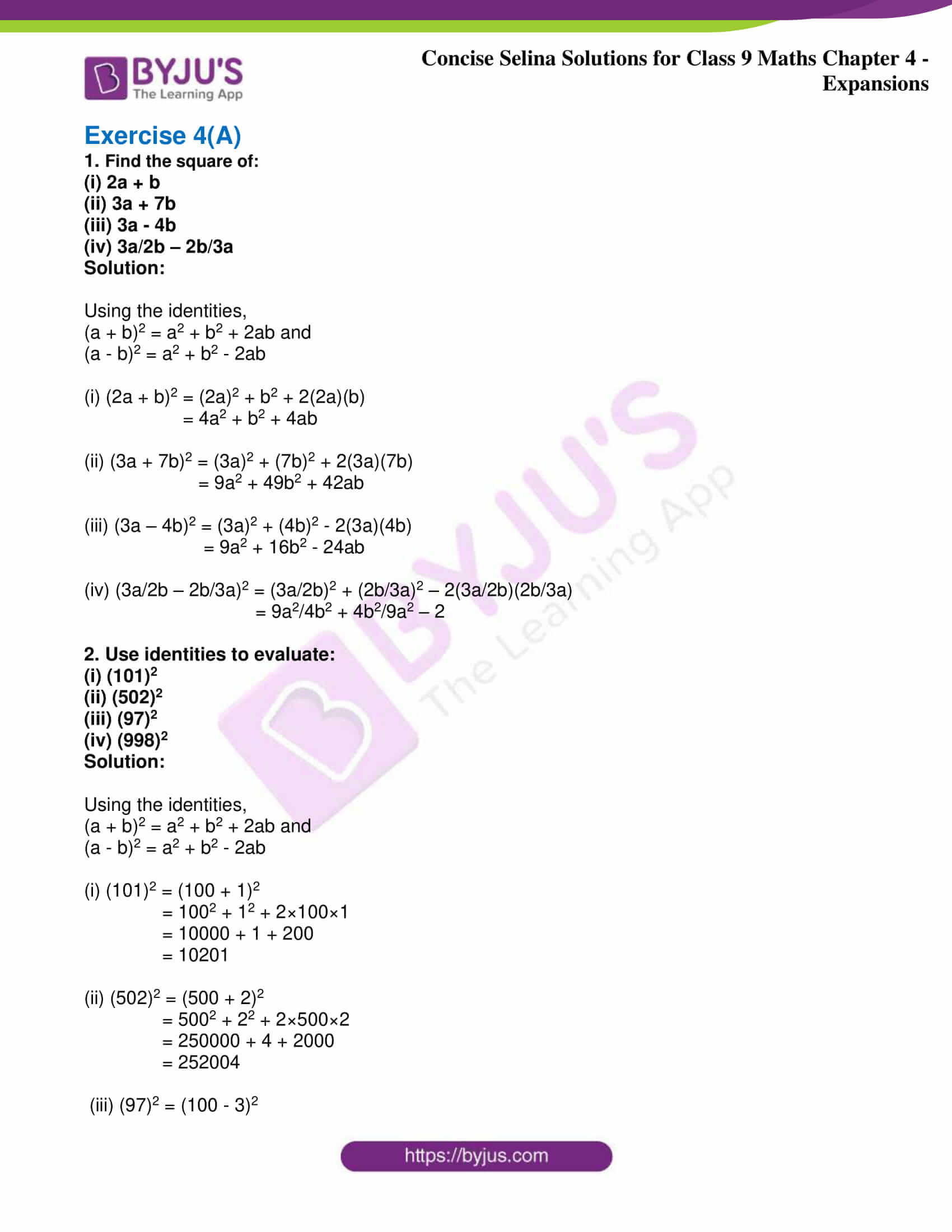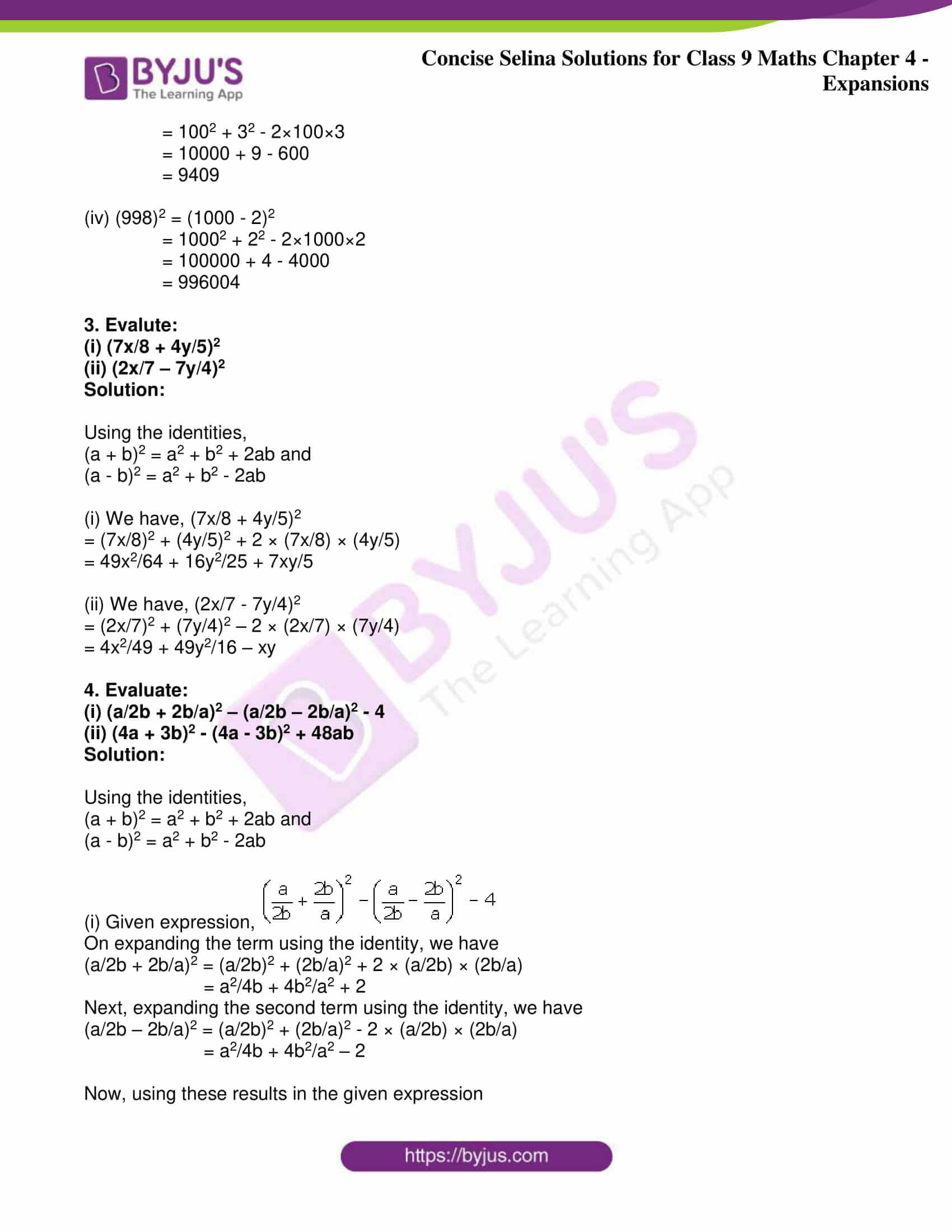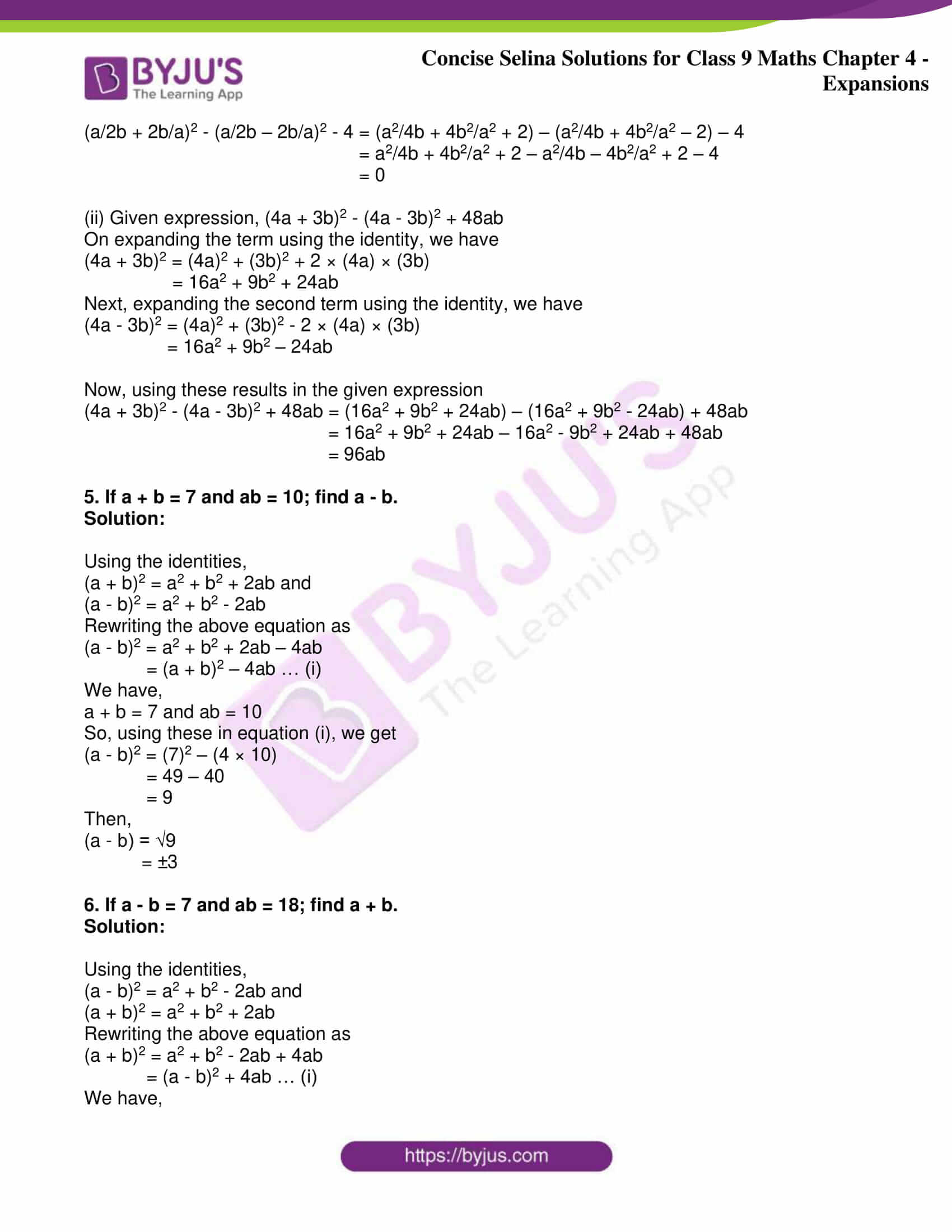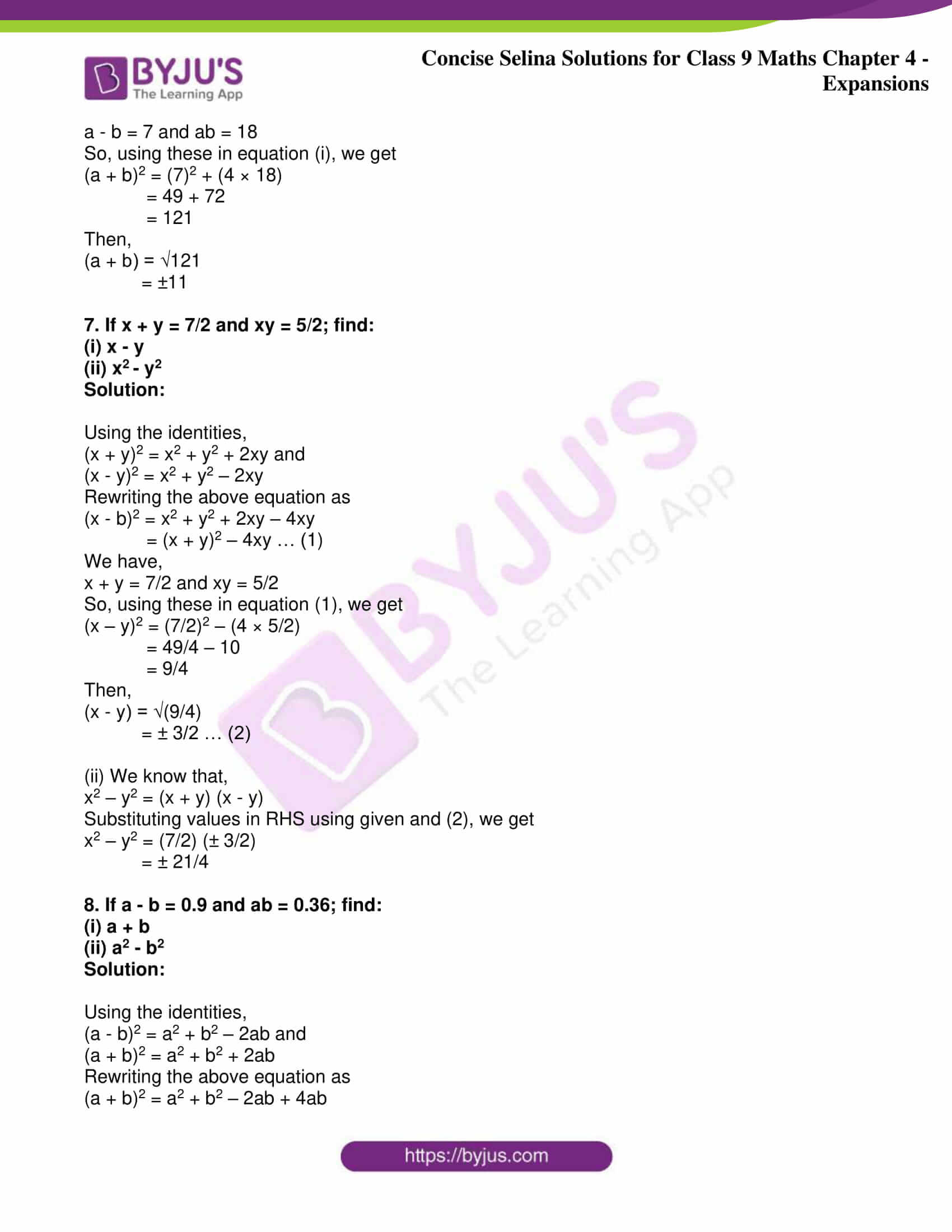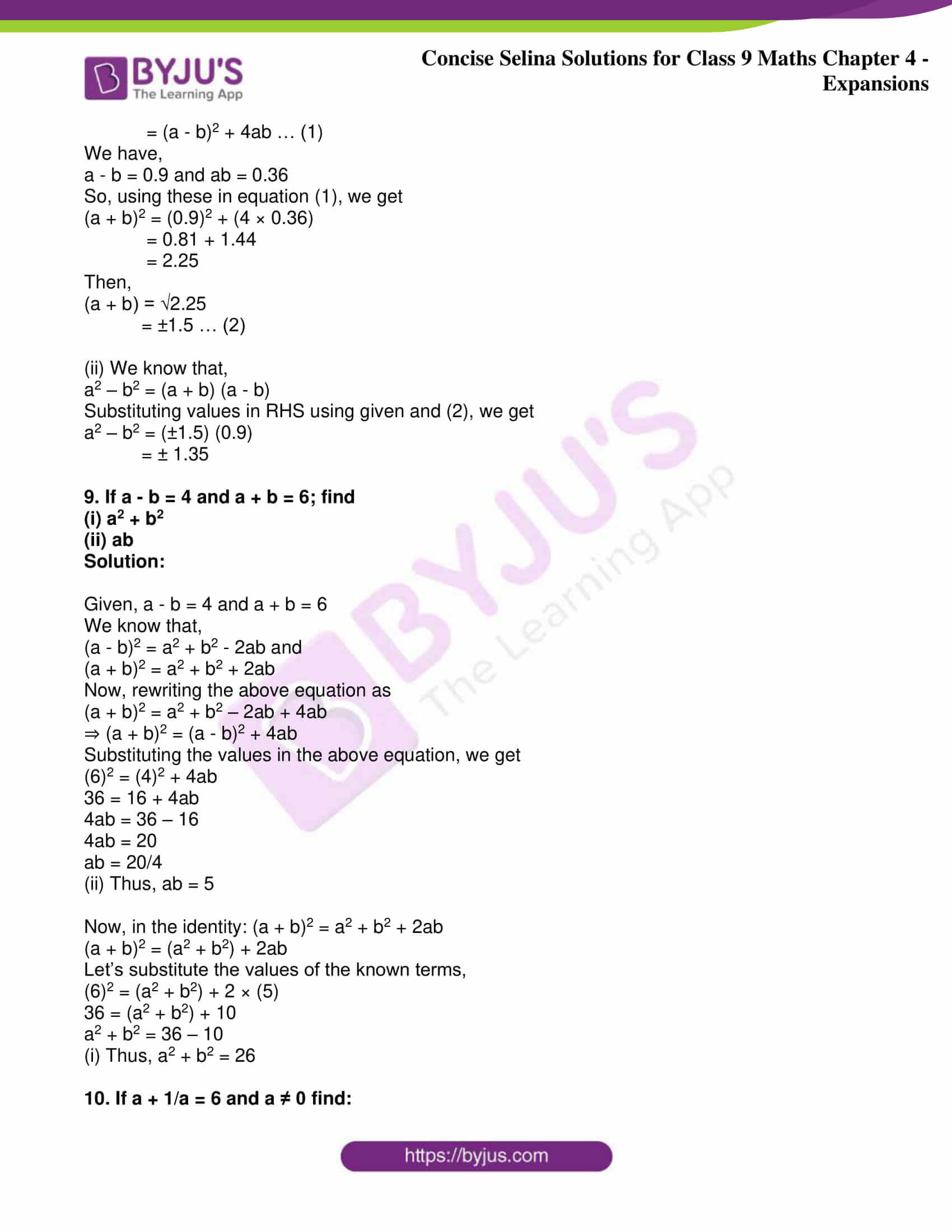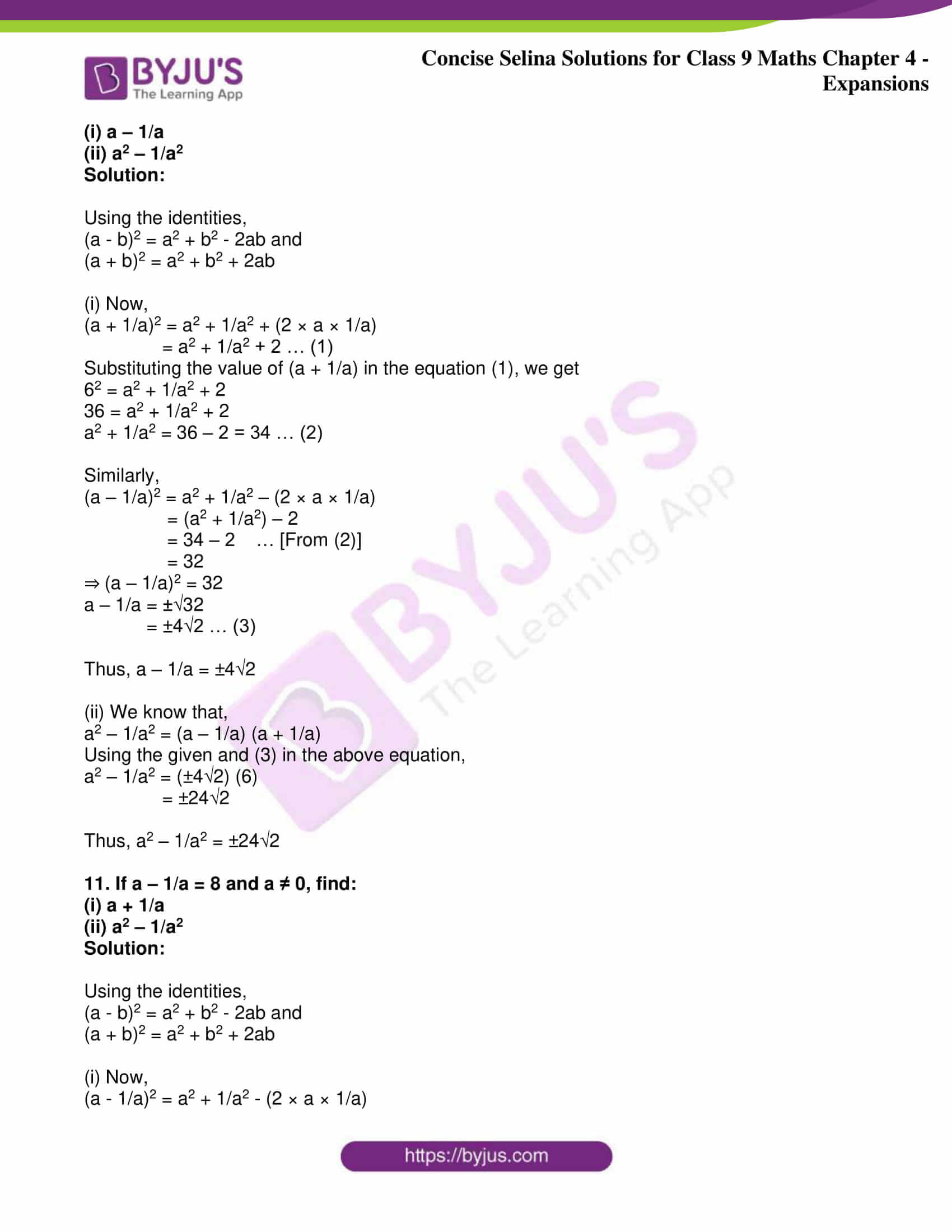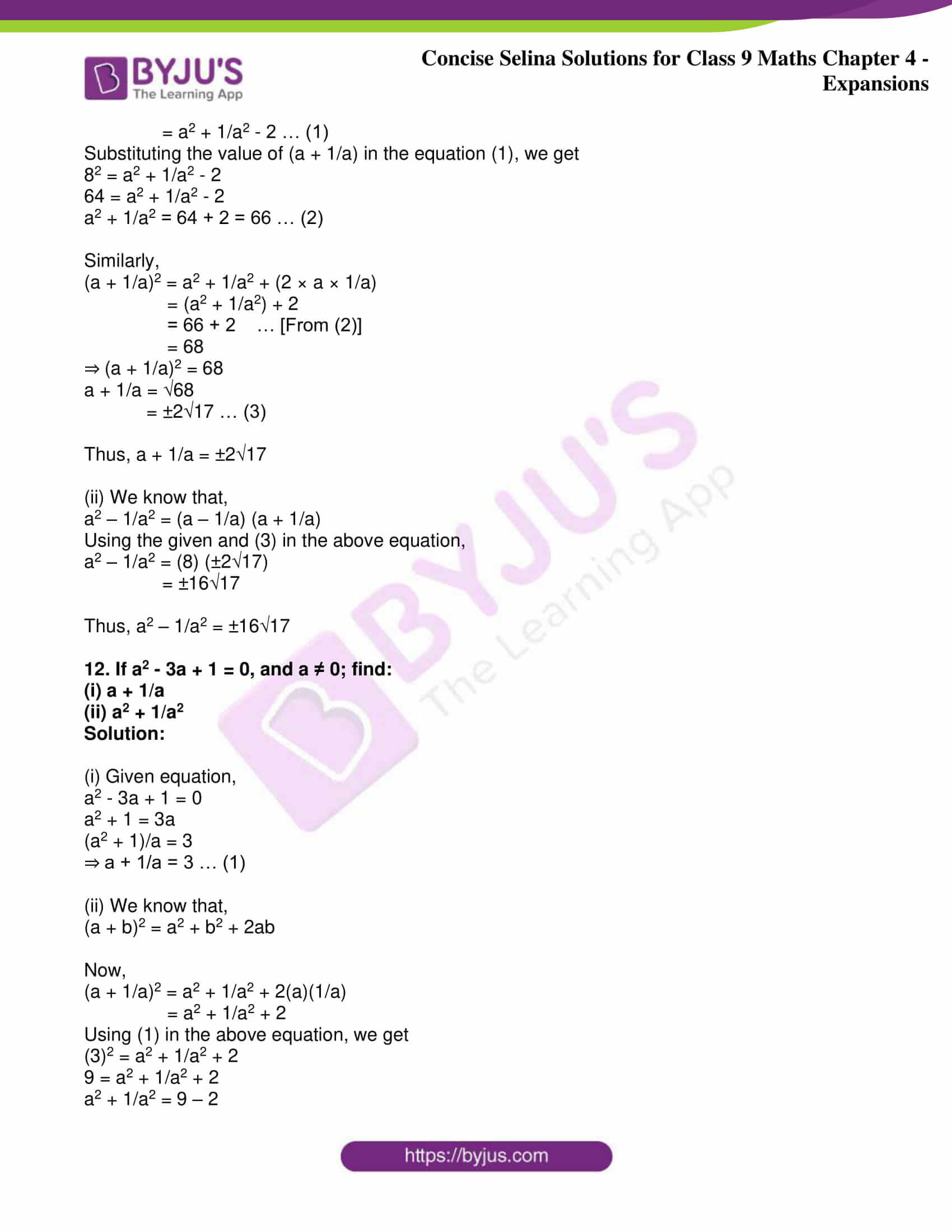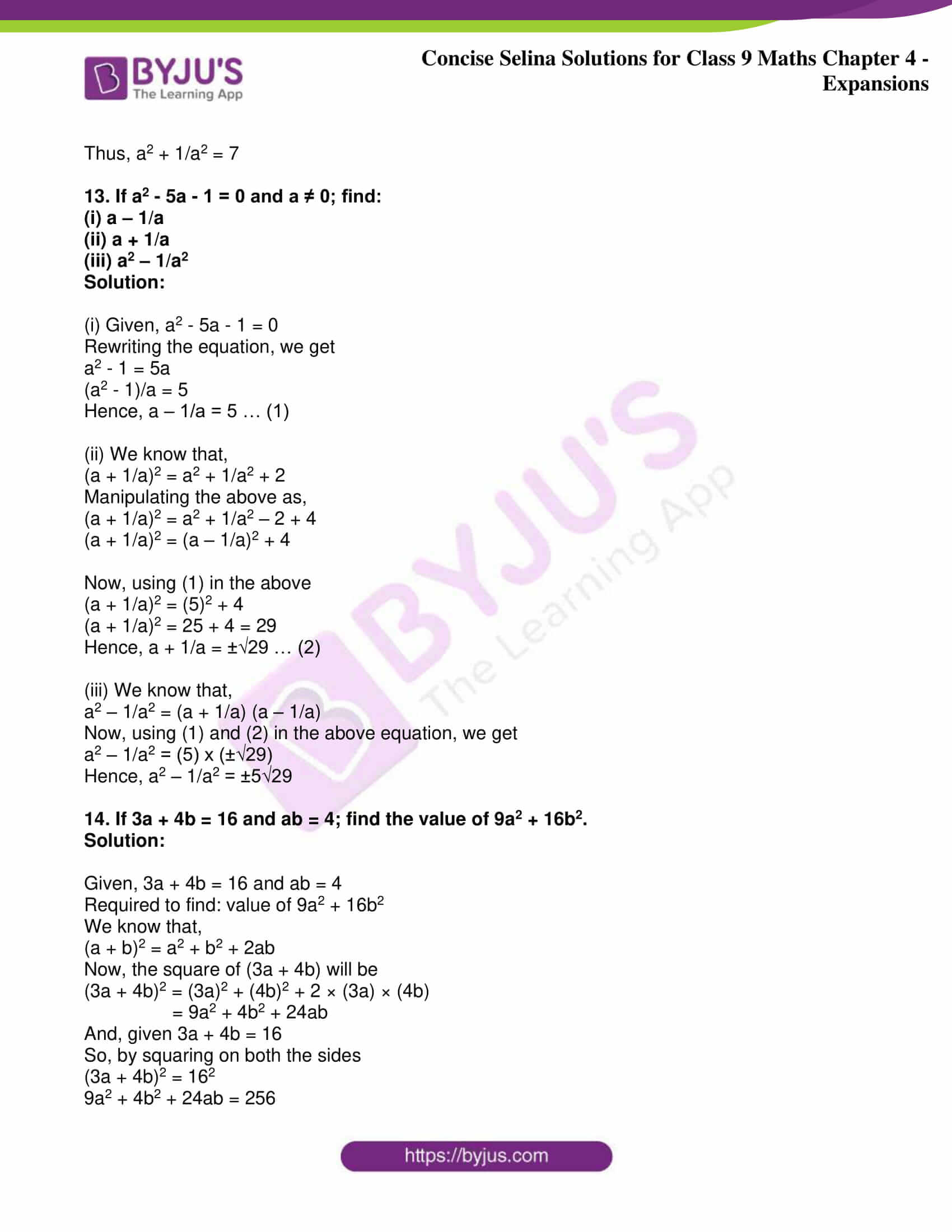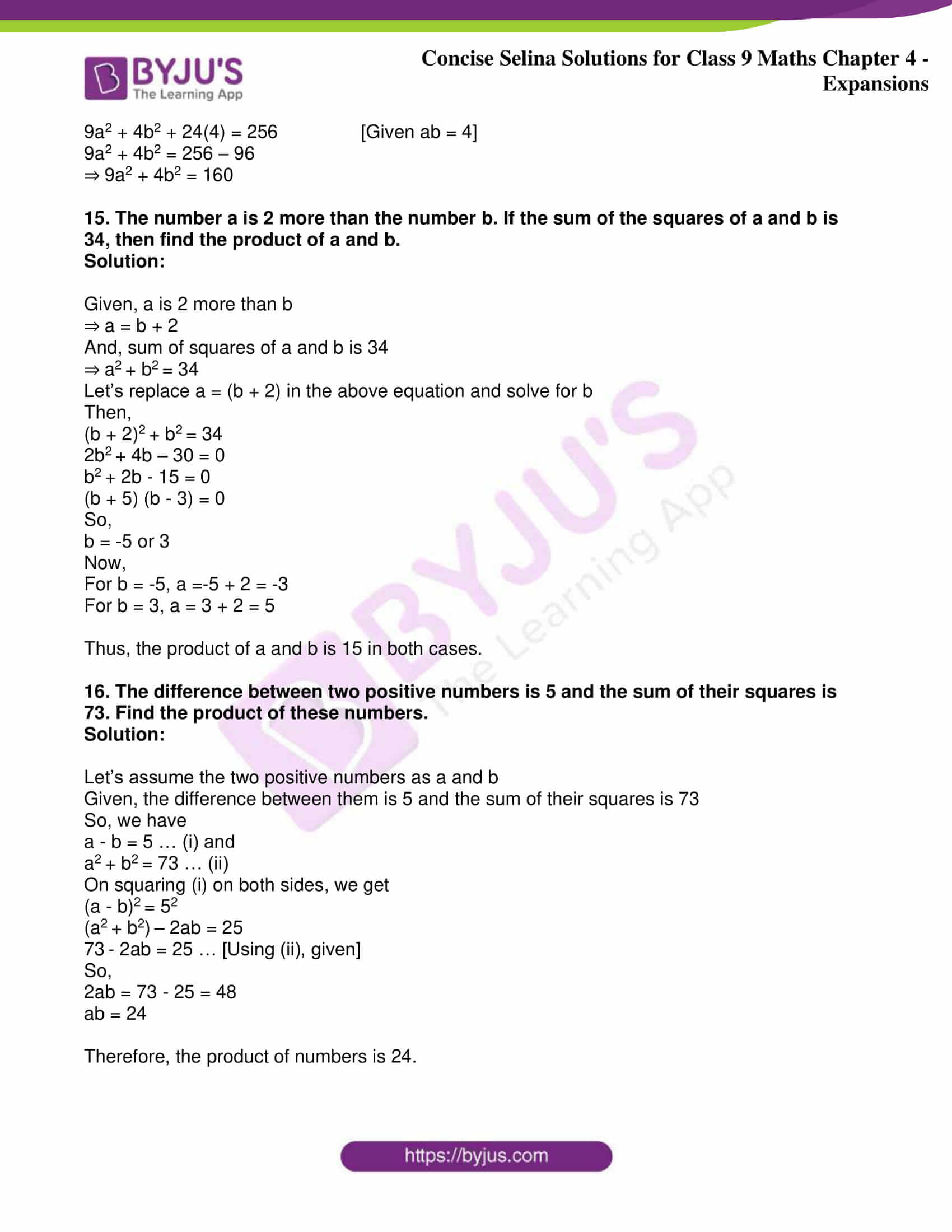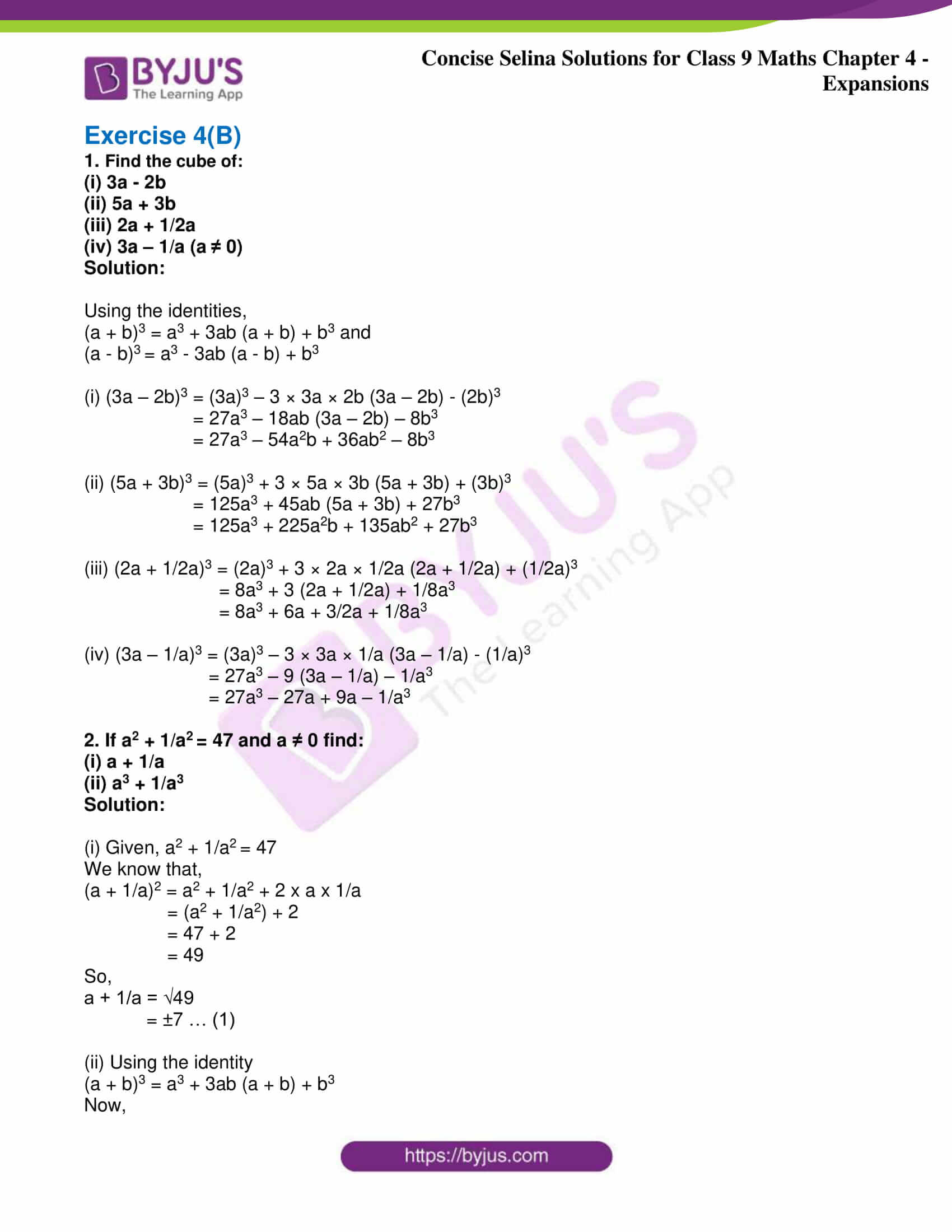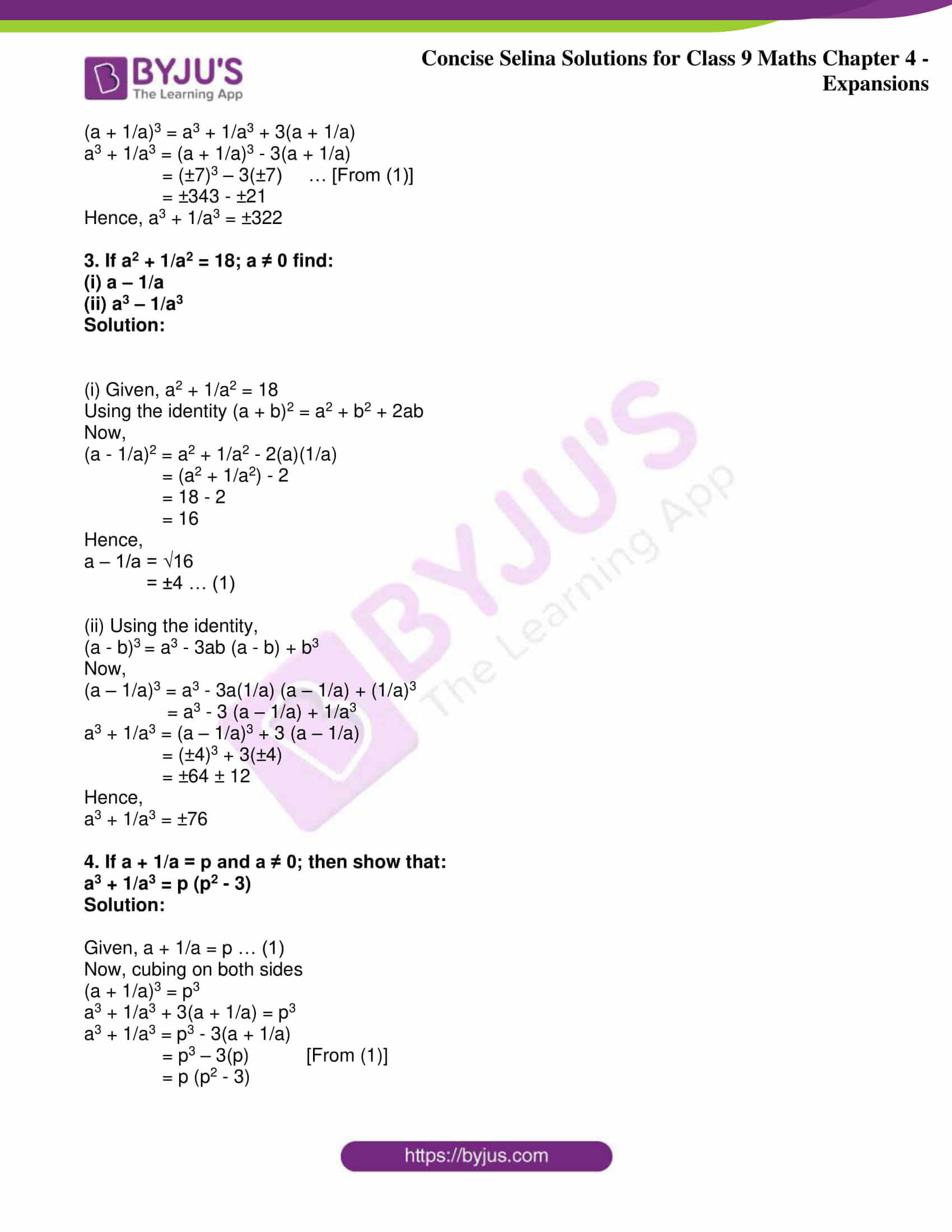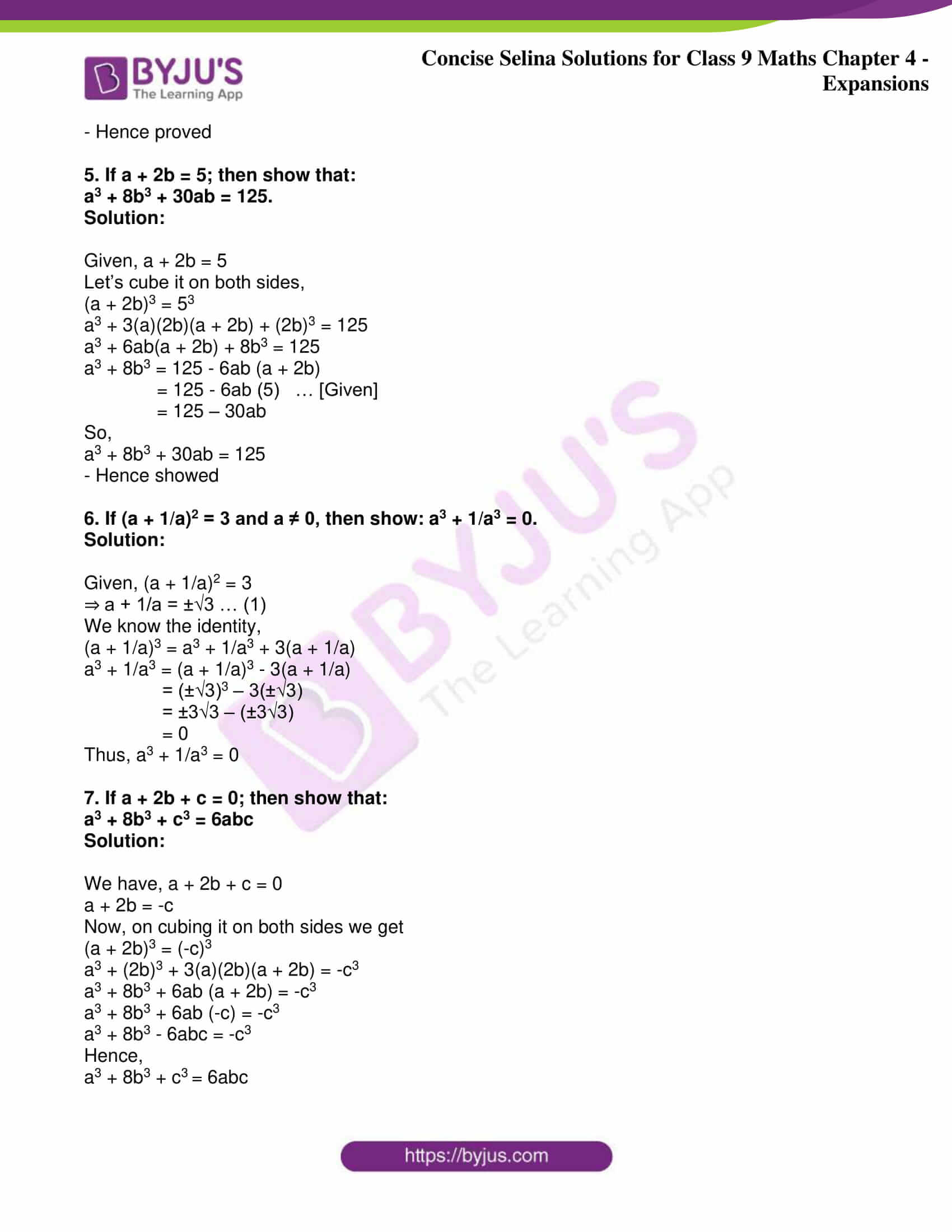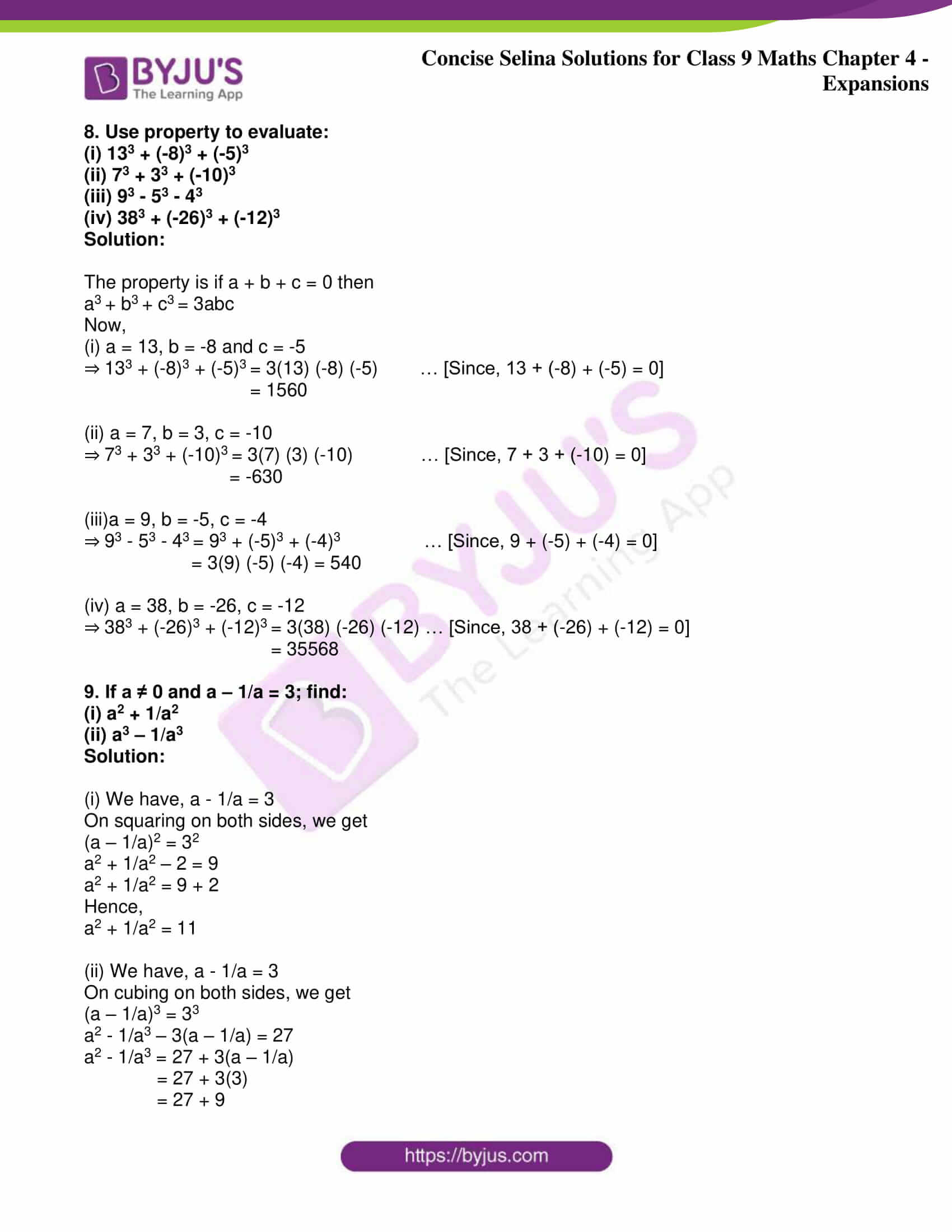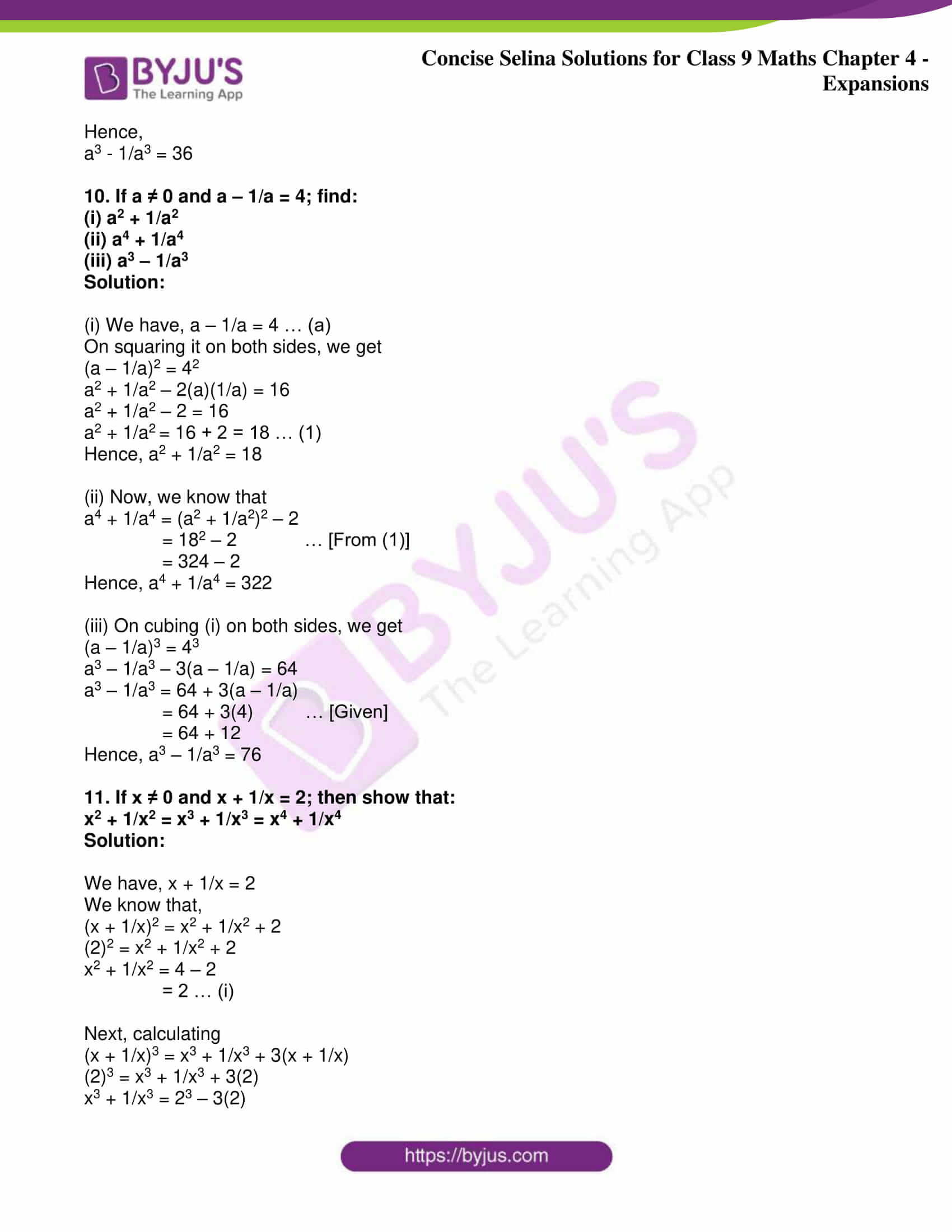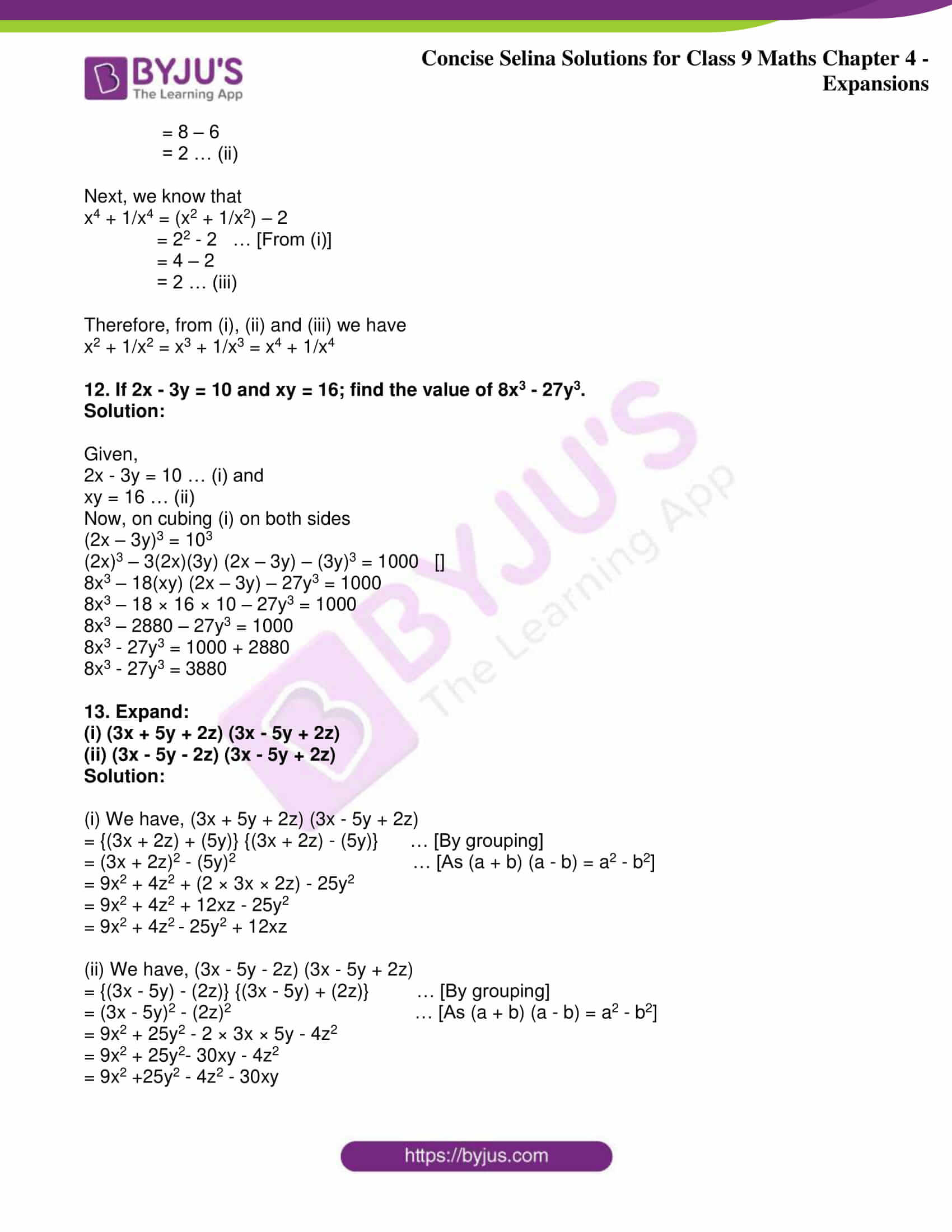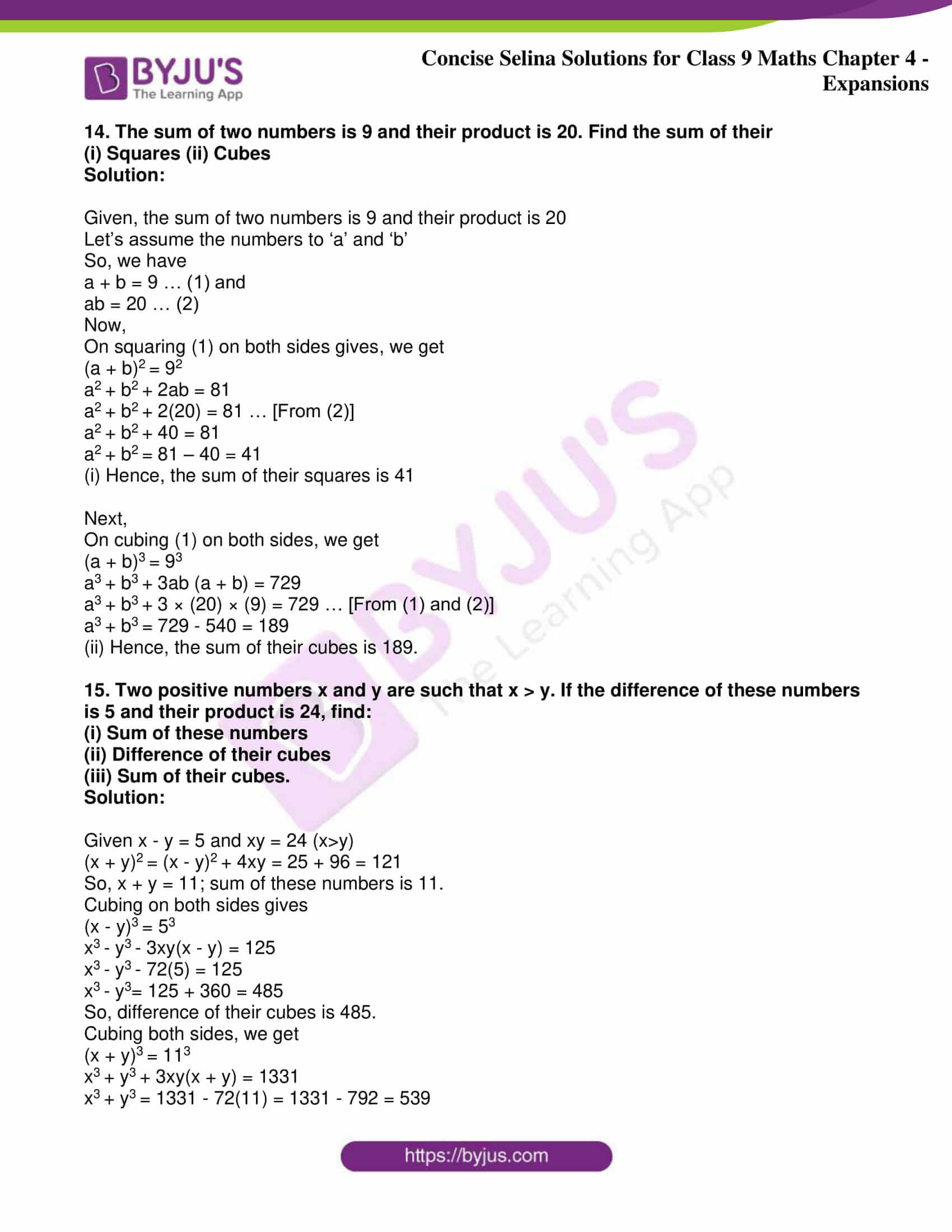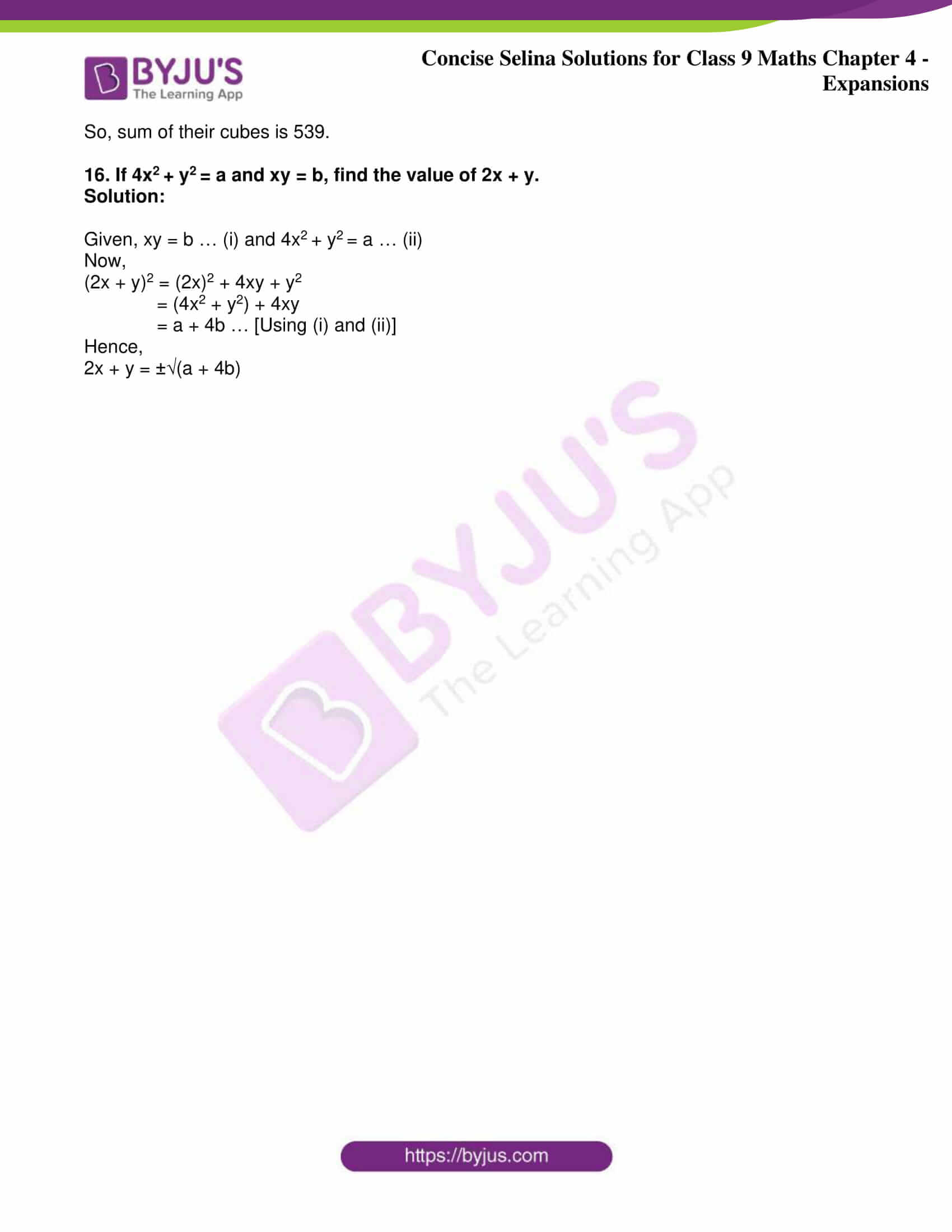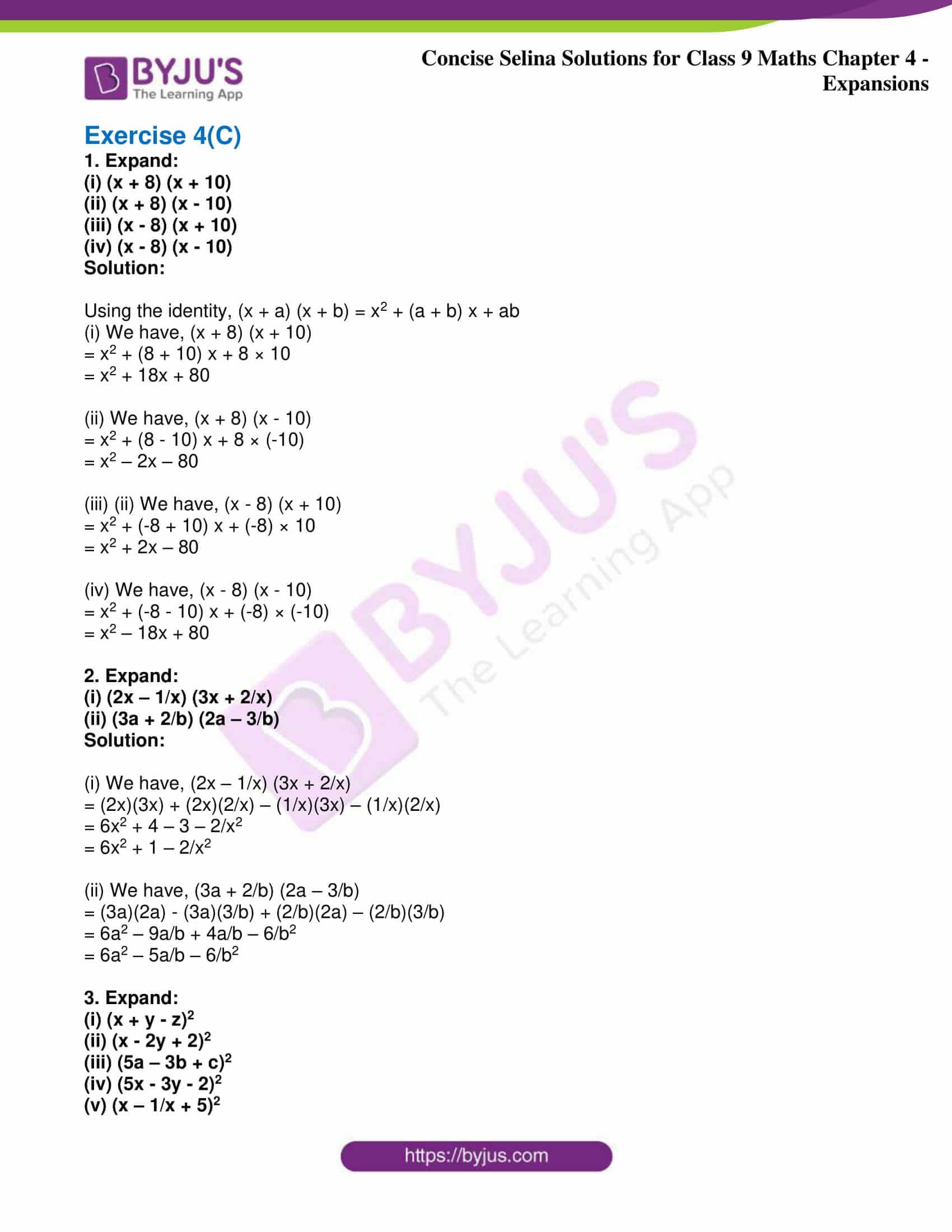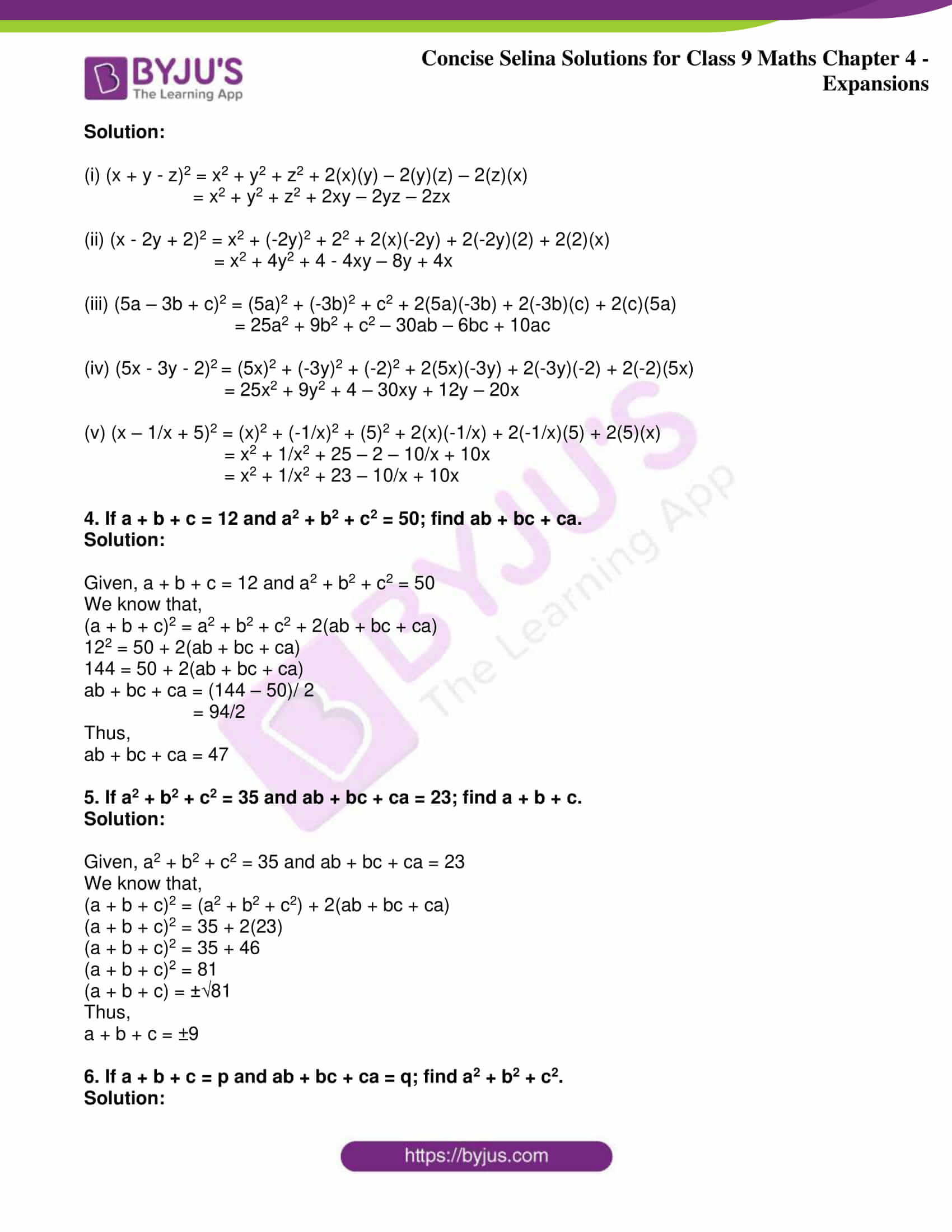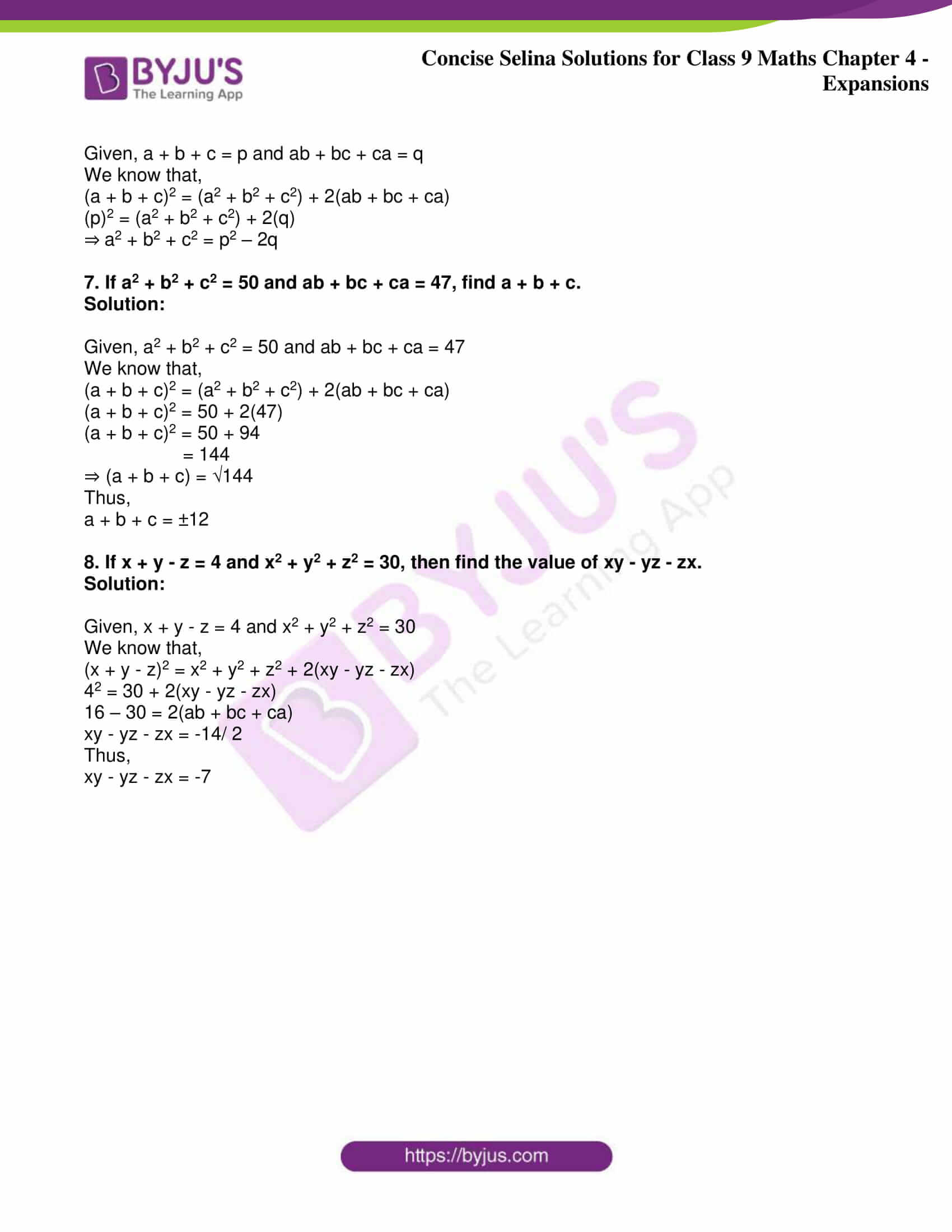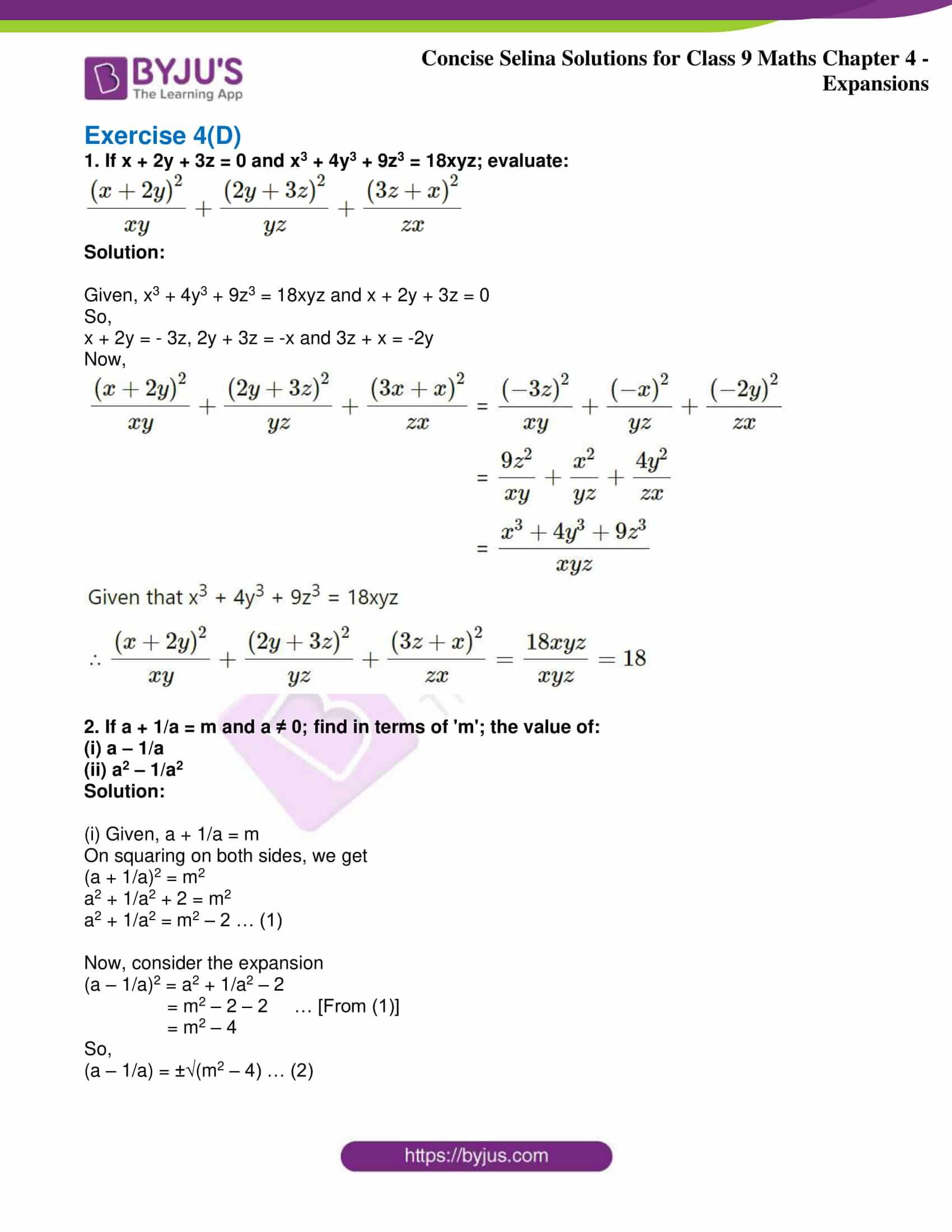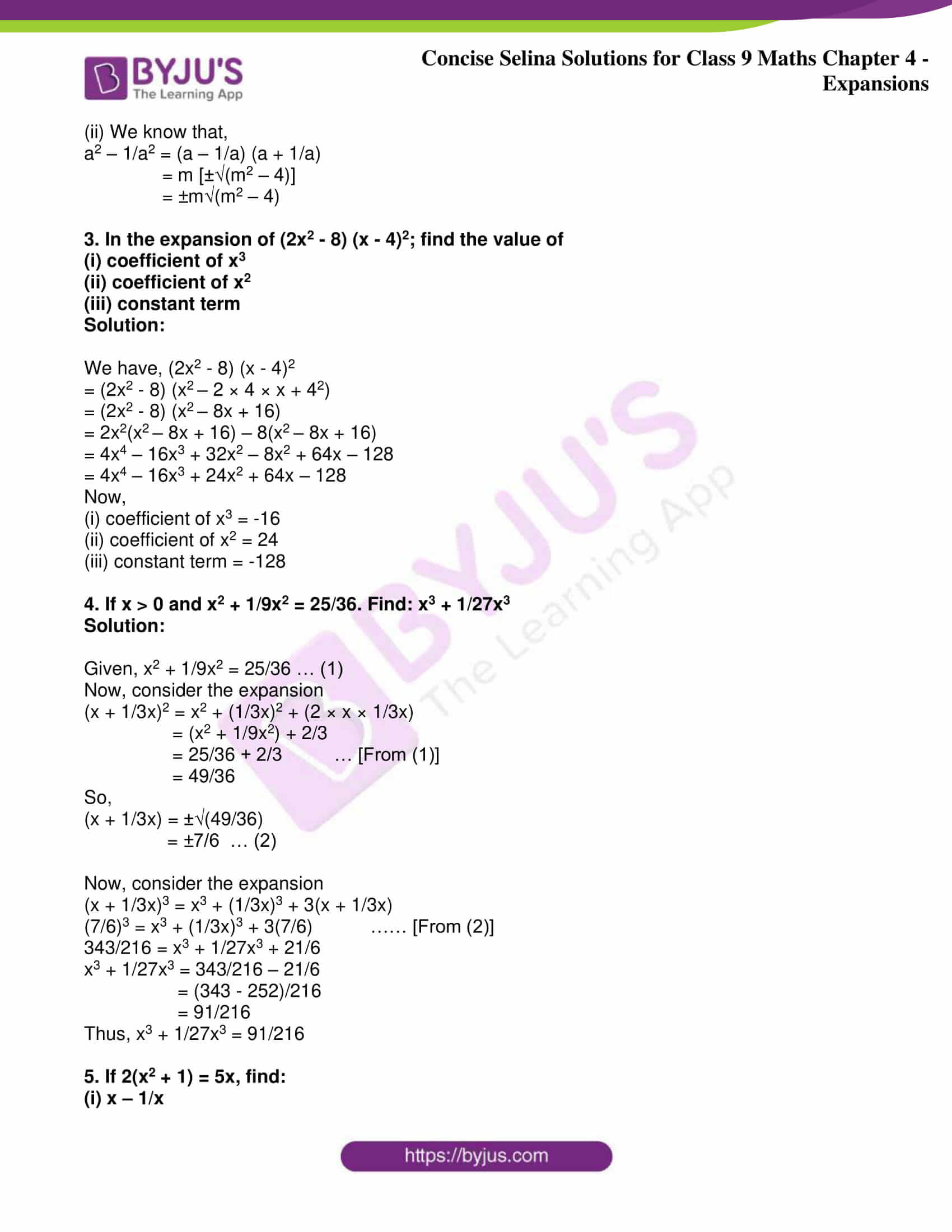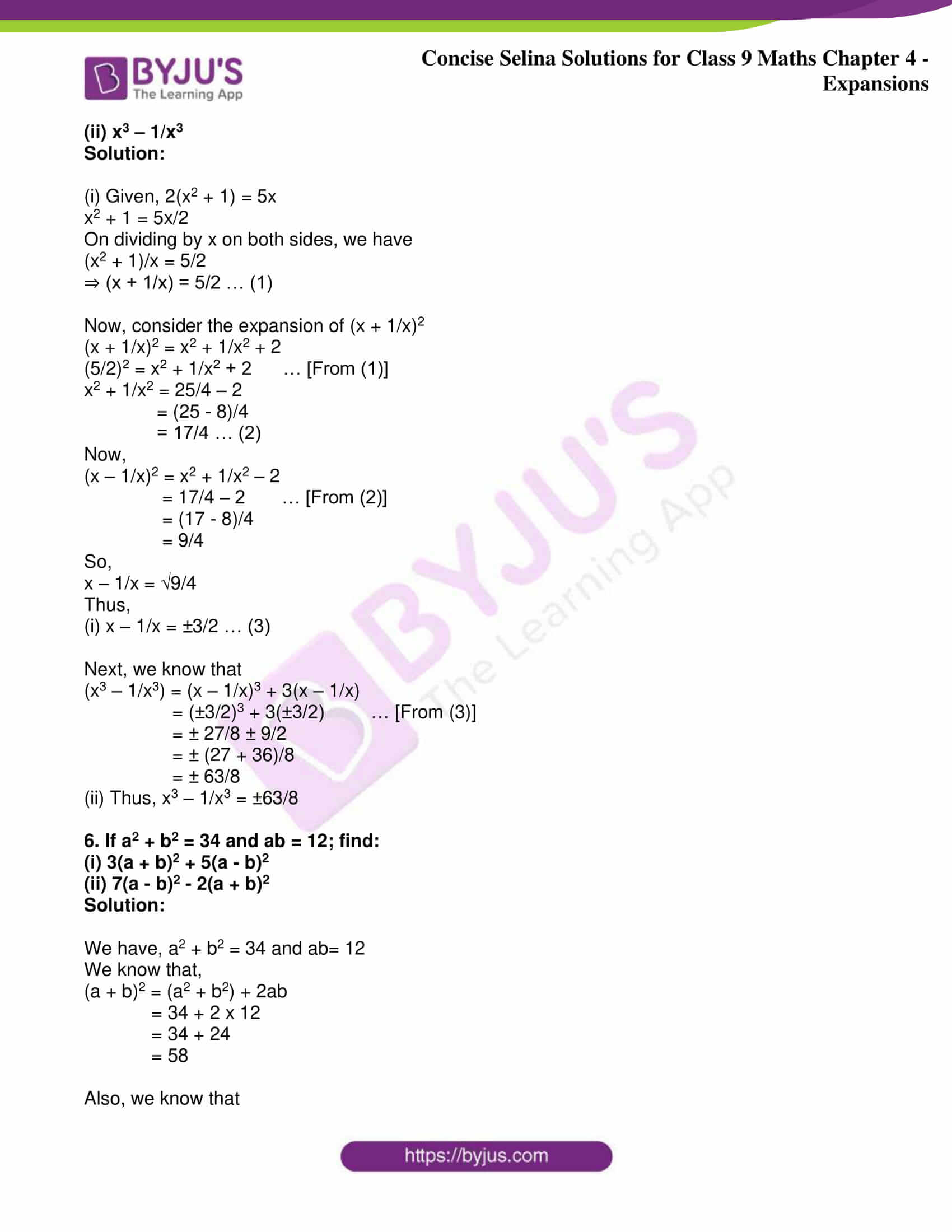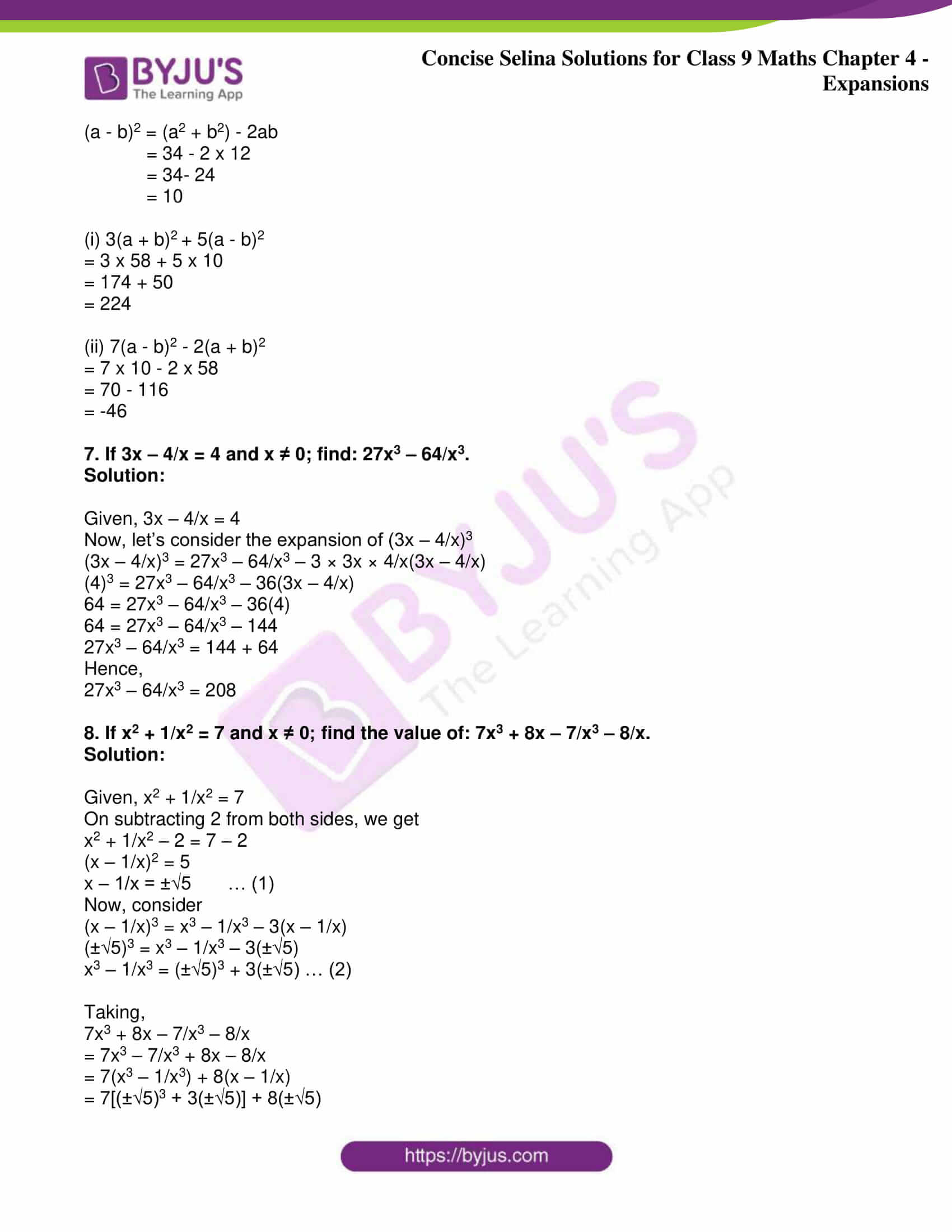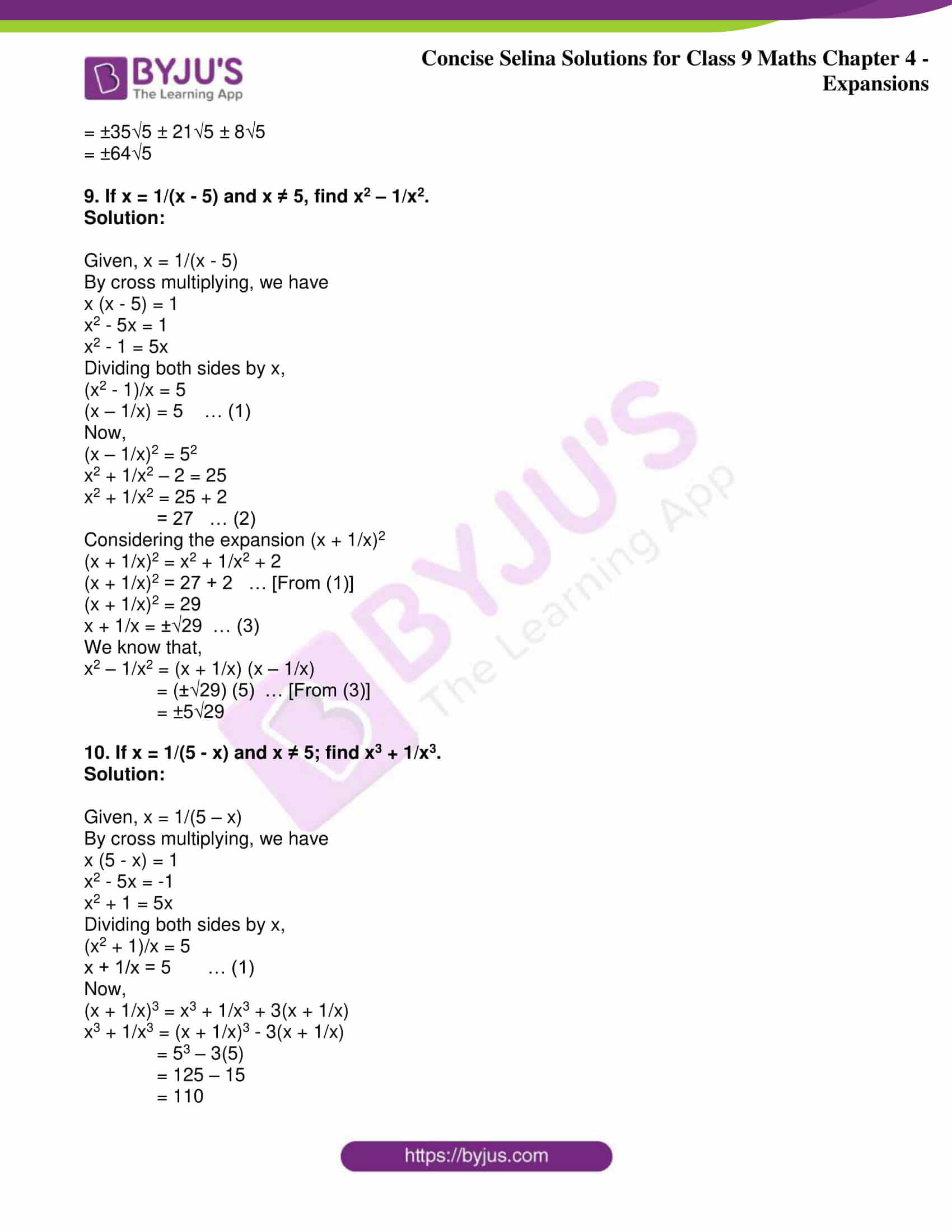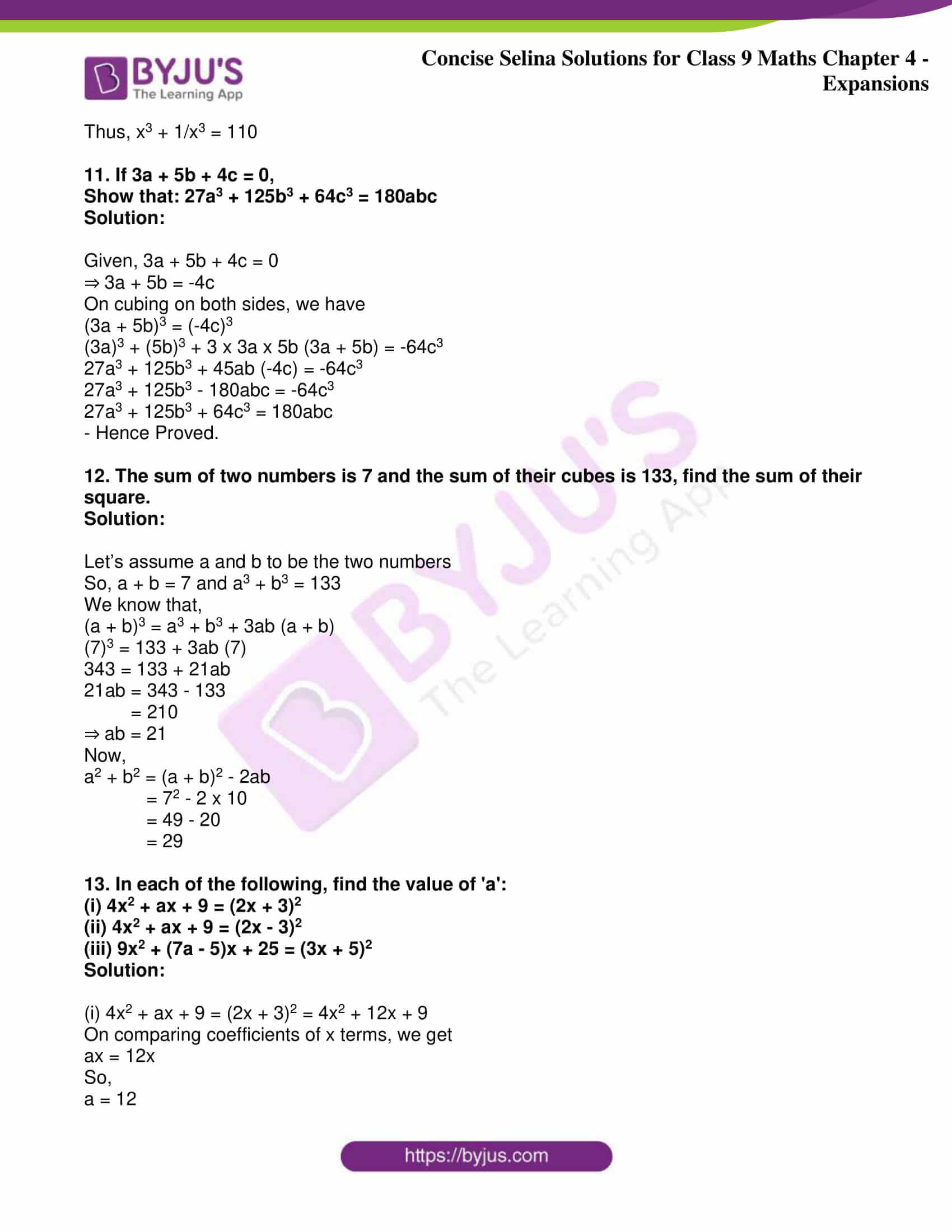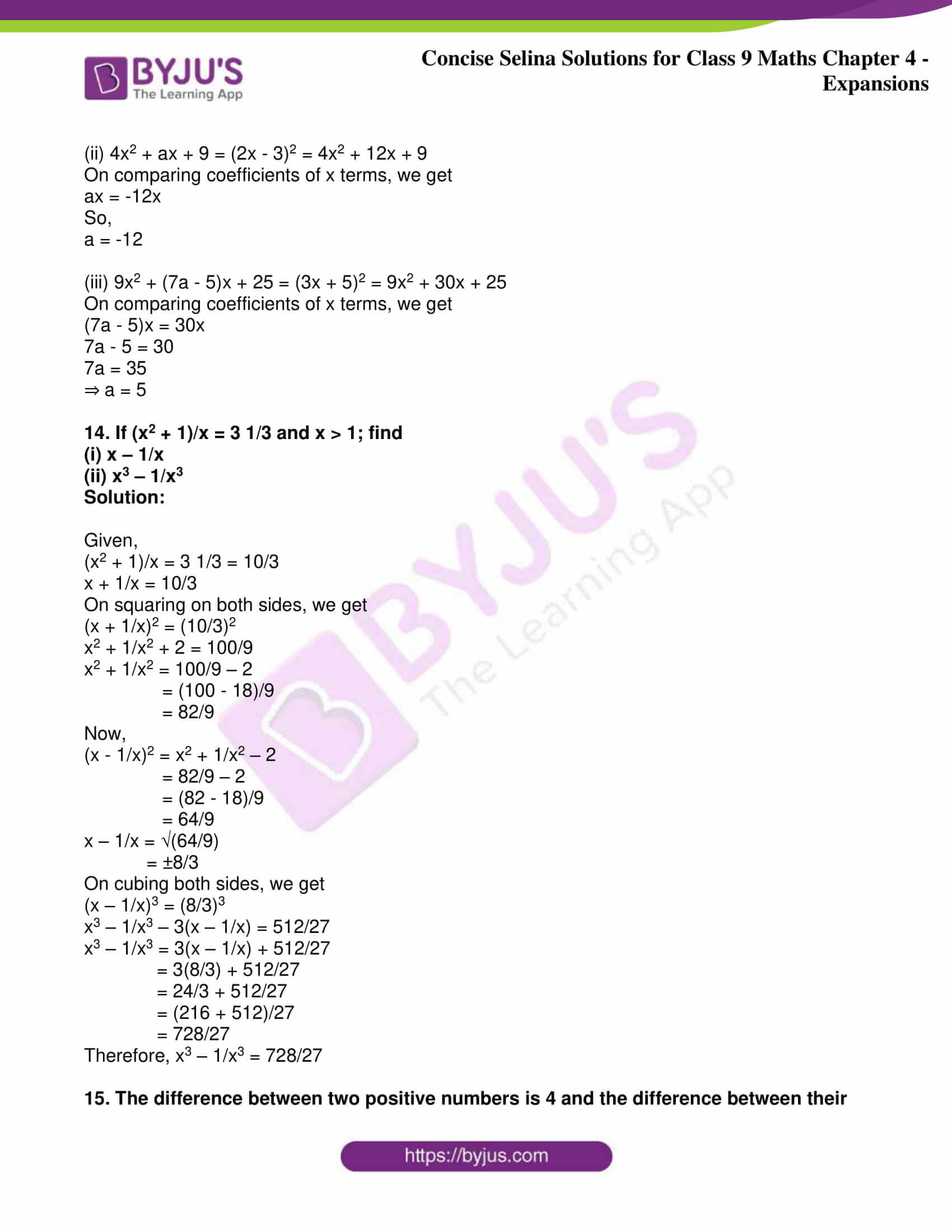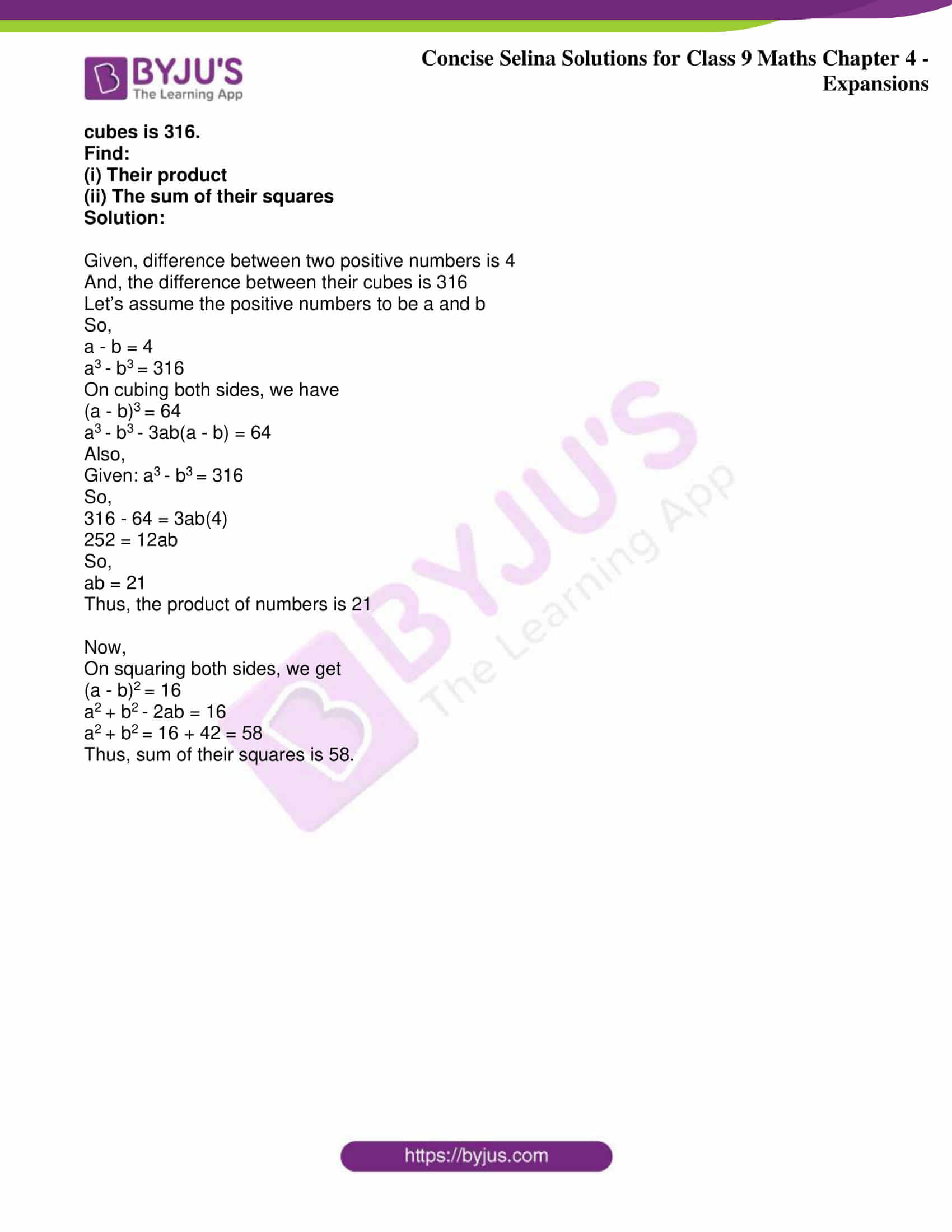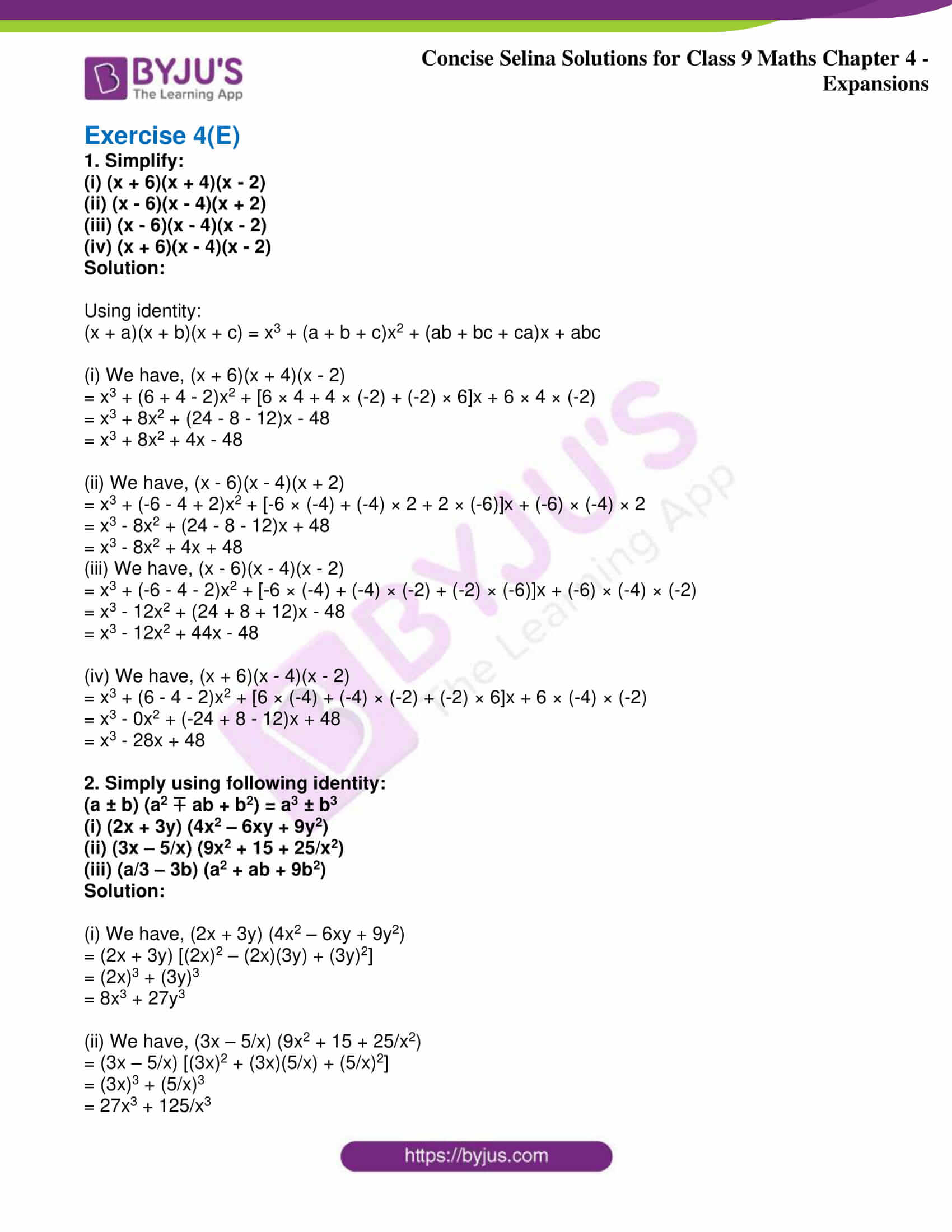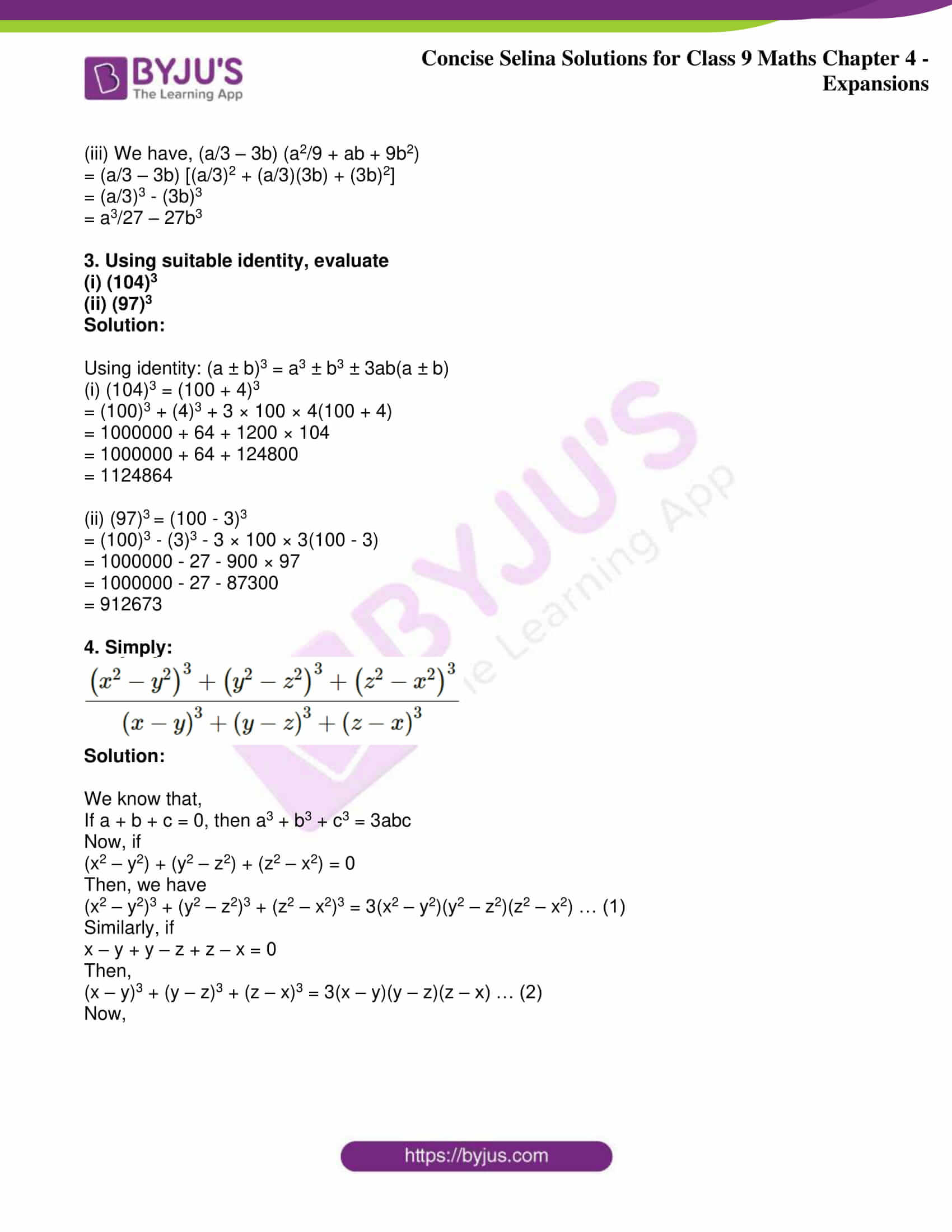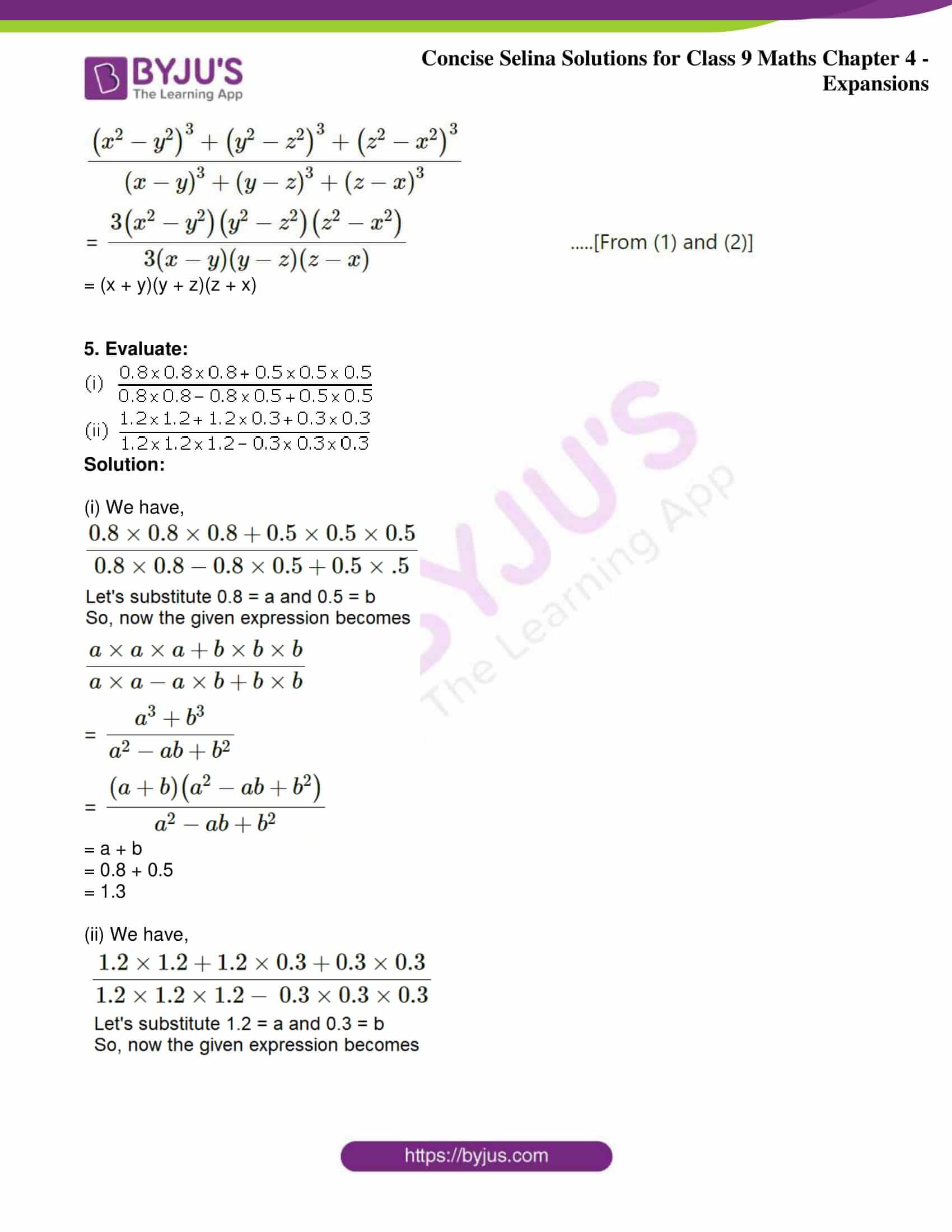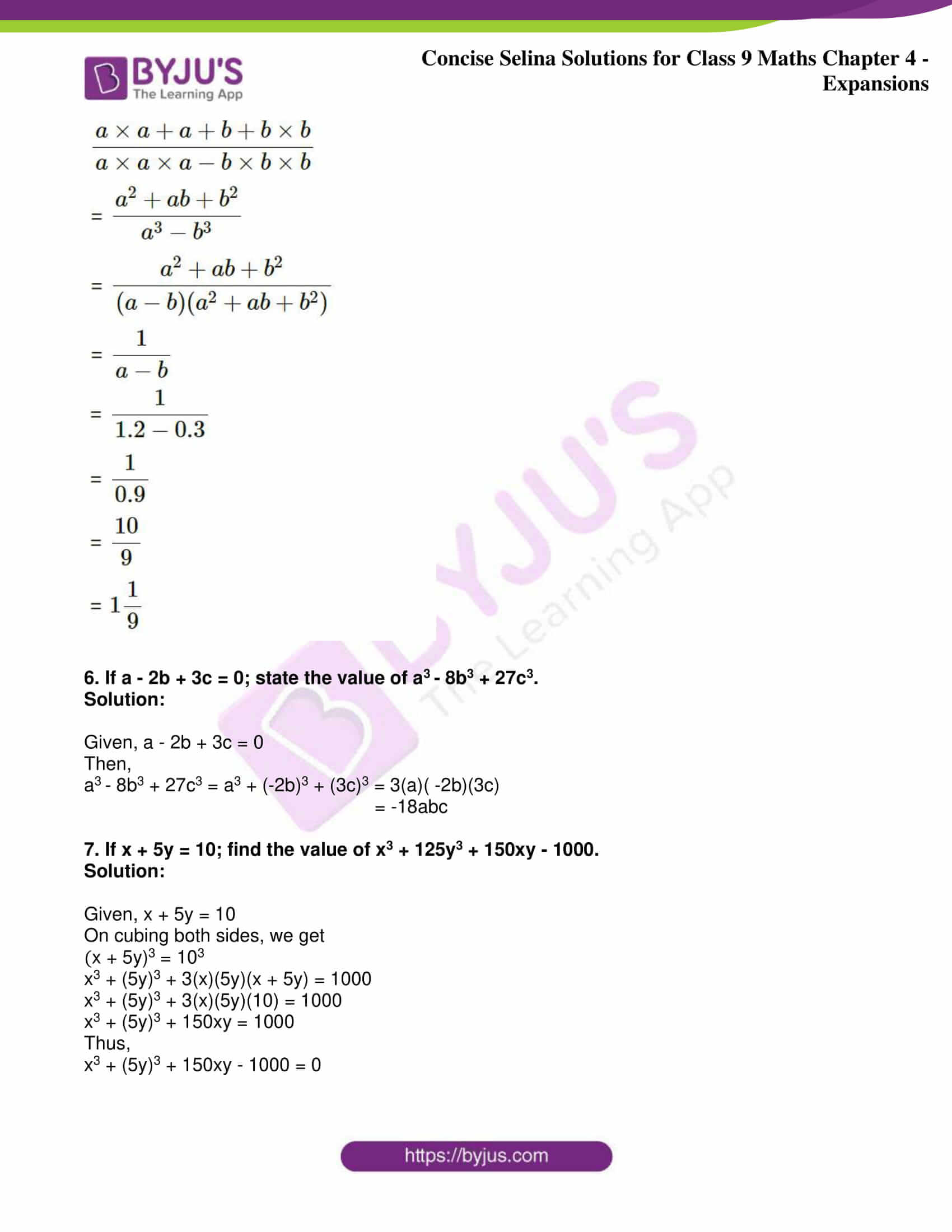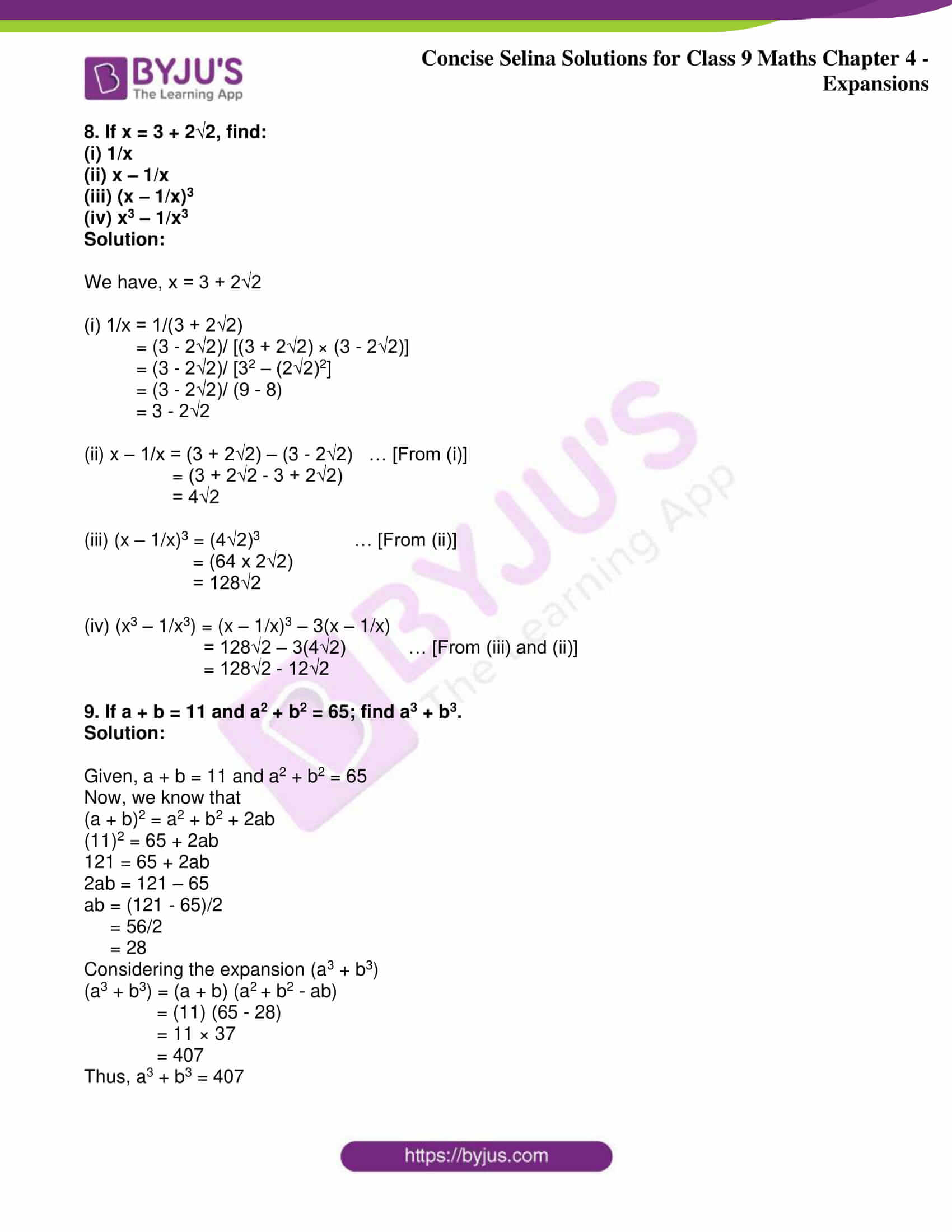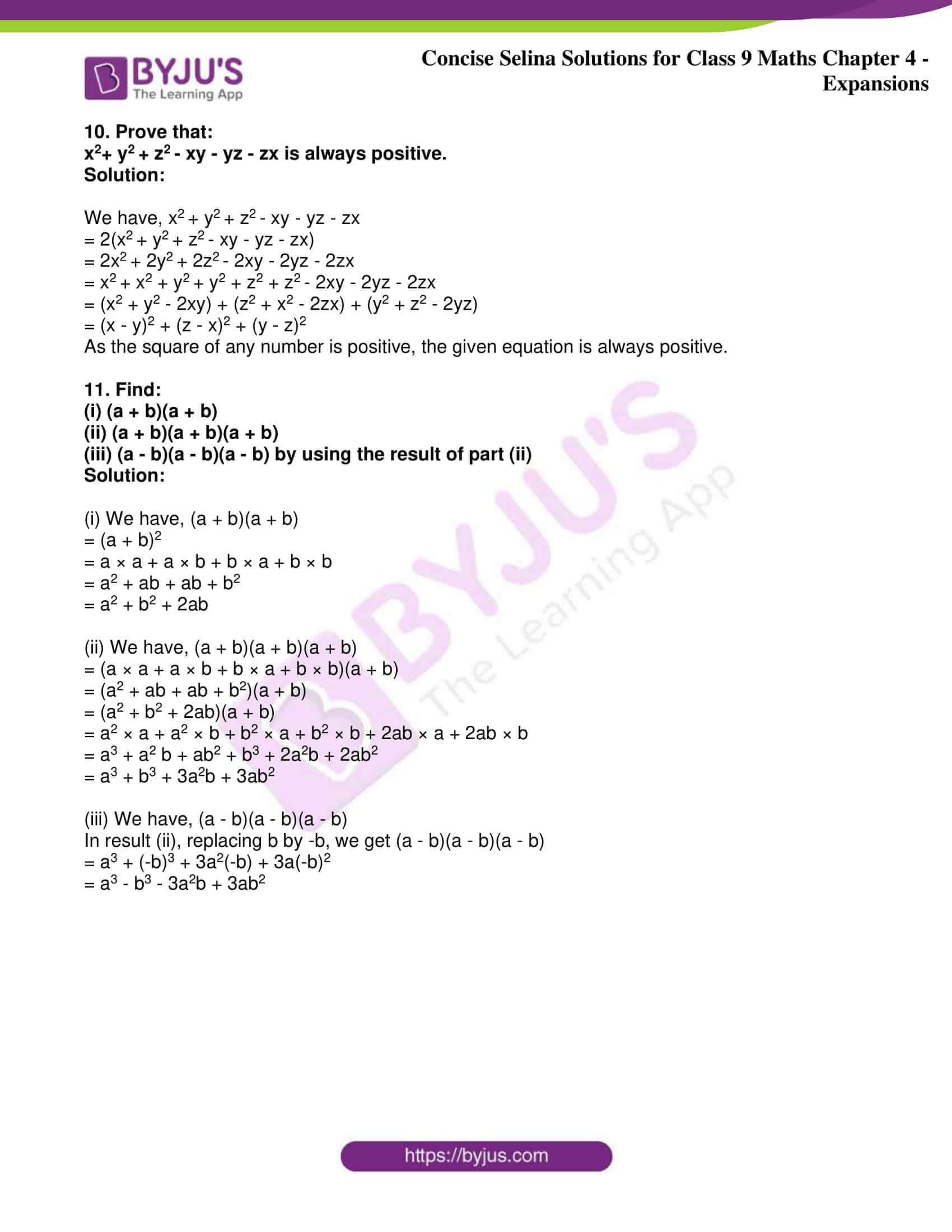Exercise 4(A)

1. Find the square of:

(i) 2a + b

(ii) 3a + 7b

(iii) 3a – 4b

(iv) 3a/2b – 2b/3a

Solution:

Using the identities,

(a + b)2 = a2 + b2 + 2ab and

(a – b)2 = a2 + b2 – 2ab

(i) (2a + b)2 = (2a)2 + b2 + 2(2a)(b)

= 4a2 + b2 + 4ab

(ii) (3a + 7b)2 = (3a)2 + (7b)2 + 2(3a)(7b)

= 9a2 + 49b2 + 42ab

(iii) (3a – 4b)2 = (3a)2 + (4b)2 – 2(3a)(4b)

= 9a2 + 16b2 – 24ab

(iv) (3a/2b – 2b/3a)2 = (3a/2b)2 + (2b/3a)2 – 2(3a/2b)(2b/3a)

= 9a2/4b2 + 4b2/9a2 – 2

2. Use identities to evaluate:

(i) (101)2

(ii) (502)2

(iii) (97)2

(iv) (998)2

Solution:

Using the identities,

(a + b)2 = a2 + b2 + 2ab and

(a – b)2 = a2 + b2 – 2ab

(i) (101)2 = (100 + 1)2

= 1002 + 12 + 2×100×1

= 10000 + 1 + 200

= 10201

(ii) (502)2 = (500 + 2)2

= 5002 + 22 + 2×500×2

= 250000 + 4 + 2000

= 252004

(iii) (97)2 = (100 – 3)2

= 1002 + 32 – 2×100×3

= 10000 + 9 – 600

= 9409

(iv) (998)2 = (1000 – 2)2

= 10002 + 22 – 2×1000×2

= 100000 + 4 – 4000

= 996004

3. Evalute:

(i) (7x/8 + 4y/5)2

(ii) (2x/7 – 7y/4)2

Solution:

Using the identities,

(a + b)2 = a2 + b2 + 2ab and

(a – b)2 = a2 + b2 – 2ab

(i) We have, (7x/8 + 4y/5)2

= (7x/8)2 + (4y/5)2 + 2 × (7x/8) × (4y/5)

= 49x2/64 + 16y2/25 + 7xy/5

(ii) We have, (2x/7 – 7y/4)2

= (2x/7)2 + (7y/4)2 – 2 × (2x/7) × (7y/4)

= 4x2/49 + 49y2/16 – xy

4. Evaluate:

(i) (a/2b + 2b/a)2 – (a/2b – 2b/a)2 – 4

(ii) (4a + 3b)2 – (4a – 3b)2 + 48ab

Solution:

Using the identities,

(a + b)2 = a2 + b2 + 2ab and

(a – b)2 = a2 + b2 – 2ab

(i) Given expression,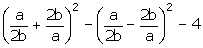On expanding the term using the identity, we have

(a/2b + 2b/a)2 = (a/2b)2 + (2b/a)2 + 2 × (a/2b) × (2b/a)

= a2/4b + 4b2/a2 + 2

Next, expanding the second term using the identity, we have

(a/2b – 2b/a)2 = (a/2b)2 + (2b/a)2 – 2 × (a/2b) × (2b/a)

= a2/4b + 4b2/a2 – 2

Now, using these results in the given expression

(a/2b + 2b/a)2 – (a/2b – 2b/a)2 – 4 = (a2/4b + 4b2/a2 + 2) – (a2/4b + 4b2/a2 – 2) – 4

= a2/4b + 4b2/a2 + 2 – a2/4b – 4b2/a2 + 2 – 4

= 0

(ii) Given expression, (4a + 3b)2 – (4a – 3b)2 + 48ab

On expanding the term using the identity, we have

(4a + 3b)2 = (4a)2 + (3b)2 + 2 × (4a) × (3b)

= 16a2 + 9b2 + 24ab

Next, expanding the second term using the identity, we have

(4a – 3b)2 = (4a)2 + (3b)2 – 2 × (4a) × (3b)

= 16a2 + 9b2 – 24ab

Now, using these results in the given expression

(4a + 3b)2 – (4a – 3b)2 + 48ab = (16a2 + 9b2 + 24ab) – (16a2 + 9b2 – 24ab) + 48ab

= 16a2 + 9b2 + 24ab – 16a2 – 9b2 + 24ab + 48ab

= 96ab

5. If a + b = 7 and ab = 10; find a – b.

Solution:

Using the identities,

(a + b)2 = a2 + b2 + 2ab and

(a – b)2 = a2 + b2 – 2ab

Rewriting the above equation as

(a – b)2 = a2 + b2 + 2ab – 4ab

= (a + b)2 – 4ab … (i)

We have,

a + b = 7 and ab = 10

So, using these in equation (i), we get

(a – b)2 = (7)2 – (4 × 10)

= 49 – 40

= 9

Then,

(a – b) = √9

= ±3

6. If a – b = 7 and ab = 18; find a + b.

Solution:

Using the identities,

(a – b)2 = a2 + b2 – 2ab and

(a + b)2 = a2 + b2 + 2ab

Rewriting the above equation as

(a + b)2 = a2 + b2 – 2ab + 4ab

= (a – b)2 + 4ab … (i)

We have,

a – b = 7 and ab = 18

So, using these in equation (i), we get

(a + b)2 = (7)2 + (4 × 18)

= 49 + 72

= 121

Then,

(a + b) = √121

= ±11

7. If x + y = 7/2 and xy = 5/2; find:

(i) x – y

(ii) x2 – y2

Solution:

Using the identities,

(x + y)2 = x2 + y2 + 2xy and

(x – y)2 = x2 + y2 – 2xy

Rewriting the above equation as

(x – b)2 = x2 + y2 + 2xy – 4xy

= (x + y)2 – 4xy … (1)

We have,

x + y = 7/2 and xy = 5/2

So, using these in equation (1), we get

(x – y)2 = (7/2)2 – (4 × 5/2)

= 49/4 – 10

= 9/4

Then,

(x – y) = √(9/4)

= ± 3/2 … (2)

(ii) We know that,

x2 – y2 = (x + y) (x – y)

Substituting values in RHS using given and (2), we get

x2 – y2 = (7/2) (± 3/2)

= ± 21/4

8. If a – b = 0.9 and ab = 0.36; find:

(i) a + b

(ii) a2 – b2

Solution:

Using the identities,

(a – b)2 = a2 + b2 – 2ab and

(a + b)2 = a2 + b2 + 2ab

Rewriting the above equation as

(a + b)2 = a2 + b2 – 2ab + 4ab

= (a – b)2 + 4ab … (1)

We have,

a – b = 0.9 and ab = 0.36

So, using these in equation (1), we get

(a + b)2 = (0.9)2 + (4 × 0.36)

= 0.81 + 1.44

= 2.25

Then,

(a + b) = √2.25

= ±1.5 … (2)

(ii) We know that,

a2 – b2 = (a + b) (a – b)

Substituting values in RHS using given and (2), we get

a2 – b2 = (±1.5) (0.9)

= ± 1.35

9. If a – b = 4 and a + b = 6; find

(i) a2 + b2

(ii) ab

Solution:

Given, a – b = 4 and a + b = 6

We know that,

(a – b)2 = a2 + b2 – 2ab and

(a + b)2 = a2 + b2 + 2ab

Now, rewriting the above equation as

(a + b)2 = a2 + b2 – 2ab + 4ab

⇒ (a + b)2 = (a – b)2 + 4ab

Substituting the values in the above equation, we get

(6)2 = (4)2 + 4ab

36 = 16 + 4ab

4ab = 36 – 16

4ab = 20

ab = 20/4

(ii) Thus, ab = 5

Now, in the identity: (a + b)2 = a2 + b2 + 2ab

(a + b)2 = (a2 + b2) + 2ab

Let’s substitute the values of the known terms,

(6)2 = (a2 + b2) + 2 × (5)

36 = (a2 + b2) + 10

a2 + b2 = 36 – 10

(i) Thus, a2 + b2 = 26

10. If a + 1/a = 6 and a ≠ 0 find:

(i) a – 1/a

(ii) a2 – 1/a2

Solution:

Using the identities,

(a – b)2 = a2 + b2 – 2ab and

(a + b)2 = a2 + b2 + 2ab

(i) Now,

(a + 1/a)2 = a2 + 1/a2 + (2 × a × 1/a)

= a2 + 1/a2 + 2 … (1)

Substituting the value of (a + 1/a) in the equation (1), we get

62 = a2 + 1/a2 + 2

36 = a2 + 1/a2 + 2

a2 + 1/a2 = 36 – 2 = 34 … (2)

Similarly,

(a – 1/a)2 = a2 + 1/a2 – (2 × a × 1/a)

= (a2 + 1/a2) – 2

= 34 – 2 … [From (2)]

= 32

⇒ (a – 1/a)2 = 32

a – 1/a = ±√32

= ±4√2 … (3)

Thus, a – 1/a = ±4√2

(ii) We know that,

a2 – 1/a2 = (a – 1/a) (a + 1/a)

Using the given and (3) in the above equation,

a2 – 1/a2 = (±4√2) (6)

= ±24√2

Thus, a2 – 1/a2 = ±24√2

11. If a – 1/a = 8 and a ≠ 0, find:

(i) a + 1/a

(ii) a2 – 1/a2

Solution:

Using the identities,

(a – b)2 = a2 + b2 – 2ab and

(a + b)2 = a2 + b2 + 2ab

(i) Now,

(a – 1/a)2 = a2 + 1/a2 – (2 × a × 1/a)

= a2 + 1/a2 – 2 … (1)

Substituting the value of (a + 1/a) in the equation (1), we get

82 = a2 + 1/a2 – 2

64 = a2 + 1/a2 – 2

a2 + 1/a2 = 64 + 2 = 66 … (2)

Similarly,

(a + 1/a)2 = a2 + 1/a2 + (2 × a × 1/a)

= (a2 + 1/a2) + 2

= 66 + 2 … [From (2)]

= 68

⇒ (a + 1/a)2 = 68

a + 1/a = √68

= ±2√17 … (3)

Thus, a + 1/a = ±2√17

(ii) We know that,

a2 – 1/a2 = (a – 1/a) (a + 1/a)

Using the given and (3) in the above equation,

a2 – 1/a2 = (8) (±2√17)

= ±16√17

Thus, a2 – 1/a2 = ±16√17

12. If a2 – 3a + 1 = 0, and a ≠ 0; find:

(i) a + 1/a

(ii) a2 + 1/a2

Solution:

(i) Given equation,

a2 – 3a + 1 = 0

a2 + 1 = 3a

(a2 + 1)/a = 3

⇒ a + 1/a = 3 … (1)

(ii) We know that,

(a + b)2 = a2 + b2 + 2ab

Now,

(a + 1/a)2 = a2 + 1/a2 + 2(a)(1/a)

= a2 + 1/a2 + 2

Using (1) in the above equation, we get

(3)2 = a2 + 1/a2 + 2

9 = a2 + 1/a2 + 2

a2 + 1/a2 = 9 – 2

Thus, a2 + 1/a2 = 7

13. If a2 – 5a – 1 = 0 and a ≠ 0; find:

(i) a – 1/a

(ii) a + 1/a

(iii) a2 – 1/a2

Solution:

(i) Given, a2 – 5a – 1 = 0

Rewriting the equation, we get

a2 – 1 = 5a

(a2 – 1)/a = 5

Hence, a – 1/a = 5 … (1)

(ii) We know that,

(a + 1/a)2 = a2 + 1/a2 + 2

Manipulating the above as,

(a + 1/a)2 = a2 + 1/a2 – 2 + 4

(a + 1/a)2 = (a – 1/a)2 + 4

Now, using (1) in the above

(a + 1/a)2 = (5)2 + 4

(a + 1/a)2 = 25 + 4 = 29

Hence, a + 1/a = ±√29 … (2)

(iii) We know that,

a2 – 1/a2 = (a + 1/a) (a – 1/a)

Now, using (1) and (2) in the above equation, we get

a2 – 1/a2 = (5) x (±√29)

Hence, a2 – 1/a2 = ±5√29

14. If 3a + 4b = 16 and ab = 4; find the value of 9a2 + 16b2.

Solution:

Given, 3a + 4b = 16 and ab = 4

Required to find: value of 9a2 + 16b2

We know that,

(a + b)2 = a2 + b2 + 2ab

Now, the square of (3a + 4b) will be

(3a + 4b)2 = (3a)2 + (4b)2 + 2 × (3a) × (4b)

= 9a2 + 4b2 + 24ab

And, given 3a + 4b = 16

So, by squaring on both the sides

(3a + 4b)2 = 162

9a2 + 4b2 + 24ab = 256

9a2 + 4b2 + 24(4) = 256 [Given ab = 4]

9a2 + 4b2 = 256 – 96

⇒ 9a2 + 4b2 = 160

15. The number a is 2 more than the number b. If the sum of the squares of a and b is 34, then find the product of a and b.

Solution:

Given, a is 2 more than b

⇒ a = b + 2

And, sum of squares of a and b is 34

⇒ a+ b= 34

Let’s replace a = (b + 2) in the above equation and solve for b

Then,

(b + 2)+ b= 34

2b+ 4b – 30 = 0

b+ 2b – 15 = 0

(b + 5) (b – 3) = 0

So,

b = -5 or 3

Now,

For b = -5, a =-5 + 2 = -3

For b = 3, a = 3 + 2 = 5

Thus, the product of a and b is 15 in both cases.

16. The difference between two positive numbers is 5 and the sum of their squares is 73. Find the product of these numbers.

Solution:

Let’s assume the two positive numbers as a and b

Given, the difference between them is 5 and the sum of their squares is 73

So, we have

a – b = 5 … (i) and

a+ b= 73 … (ii)

On squaring (i) on both sides, we get

(a – b)= 52

(a+ b2) – 2ab = 25

73 – 2ab = 25 … [Using (ii), given]

So,

2ab = 73 – 25 = 48

ab = 24

Therefore, the product of numbers is 24.

Exercise 4(B)

1. Find the cube of:

(i) 3a – 2b

(ii) 5a + 3b

(iii) 2a + 1/2a

(iv) 3a – 1/a (a ≠ 0)

Solution:

Using the identities,

(a + b)3 = a3 + 3ab (a + b) + b3 and

(a – b)3 = a3 – 3ab (a – b) + b3

(i) (3a – 2b)3 = (3a)3 – 3 × 3a × 2b (3a – 2b) – (2b)3

= 27a3 – 18ab (3a – 2b) – 8b3

= 27a3 – 54a2b + 36ab2 – 8b3

(ii) (5a + 3b)3 = (5a)3 + 3 × 5a × 3b (5a + 3b) + (3b)3

= 125a3 + 45ab (5a + 3b) + 27b3

= 125a3 + 225a2b + 135ab2 + 27b3

(iii) (2a + 1/2a)3 = (2a)3 + 3 × 2a × 1/2a (2a + 1/2a) + (1/2a)3

= 8a3 + 3 (2a + 1/2a) + 1/8a3

= 8a3 + 6a + 3/2a + 1/8a3

(iv) (3a – 1/a)3 = (3a)3 – 3 × 3a × 1/a (3a – 1/a) – (1/a)3

= 27a3 – 9 (3a – 1/a) – 1/a3

= 27a3 – 27a + 9a – 1/a3

2. If a2 + 1/a2 = 47 and a ≠ 0 find:

(i) a + 1/a

(ii) a3 + 1/a3

Solution:

(i) Given, a2 + 1/a2 = 47

We know that,

(a + 1/a)2 = a2 + 1/a2 + 2 x a x 1/a

= (a2 + 1/a2) + 2

= 47 + 2

= 49

So,

a + 1/a = √49

= ±7 … (1)

(ii) Using the identity

(a + b)3 = a3 + 3ab (a + b) + b3

Now,

(a + 1/a)3 = a3 + 1/a3 + 3(a + 1/a)

a3 + 1/a3 = (a + 1/a)3 – 3(a + 1/a)

= (±7)3 – 3(±7) … [From (1)]

= ±343 – ±21

Hence, a3 + 1/a3 = ±322

3. If a2 + 1/a2 = 18; a ≠ 0 find:

(i) a – 1/a

(ii) a3 – 1/a3

Solution:

(i) Given, a2 + 1/a2 = 18

Using the identity (a + b)2 = a2 + b2 + 2ab

Now,

(a – 1/a)2 = a2 + 1/a2 – 2(a)(1/a)

= (a2 + 1/a2) – 2

= 18 – 2

= 16

Hence,

a – 1/a = √16

= ±4 … (1)

(ii) Using the identity,

(a – b)3 = a3 – 3ab (a – b) + b3

Now,

(a – 1/a)3 = a3 – 3a(1/a) (a – 1/a) + (1/a)3

= a3 – 3 (a – 1/a) + 1/a3

a3 + 1/a3 = (a – 1/a)3 + 3 (a – 1/a)

= (±4)3 + 3(±4)

= ±64 ± 12

Hence,

a3 + 1/a3 = ±76

4. If a + 1/a = p and a ≠ 0; then show that:

a3 + 1/a3 = p (p2 – 3)

Solution:

Given, a + 1/a = p … (1)

Now, cubing on both sides

(a + 1/a)3 = p3

a3 + 1/a3 + 3(a + 1/a) = p3

a3 + 1/a3 = p3 – 3(a + 1/a)

= p3 – 3(p) [From (1)]

= p (p2 – 3)

– Hence proved

5. If a + 2b = 5; then show that:

a3 + 8b3 + 30ab = 125.

Solution:

Given, a + 2b = 5

Let’s cube it on both sides,

(a + 2b)3 = 53

a3 + 3(a)(2b)(a + 2b) + (2b)3 = 125

a3 + 6ab(a + 2b) + 8b3 = 125

a3 + 8b3 = 125 – 6ab (a + 2b)

= 125 – 6ab (5) … [Given]

= 125 – 30ab

So,

a3 + 8b3 + 30ab = 125

– Hence showed

6. If (a + 1/a)2 = 3 and a ≠ 0, then show: a3 + 1/a3 = 0.

Solution:

Given, (a + 1/a)2 = 3

⇒ a + 1/a = ±√3 … (1)

We know the identity,

(a + 1/a)3 = a3 + 1/a3 + 3(a + 1/a)

a3 + 1/a3 = (a + 1/a)3 – 3(a + 1/a)

= (±√3)3 – 3(±√3)

= ±3√3 – (±3√3)

= 0

Thus, a3 + 1/a3 = 0

7. If a + 2b + c = 0; then show that:

a3 + 8b3 + c3 = 6abc

Solution:

We have, a + 2b + c = 0

a + 2b = -c

Now, on cubing it on both sides we get

(a + 2b)3 = (-c)3

a3 + (2b)3 + 3(a)(2b)(a + 2b) = -c3

a3 + 8b3 + 6ab (a + 2b) = -c3

a3 + 8b3 + 6ab (-c) = -c3

a3 + 8b3 – 6abc = -c3

Hence,

a3 + 8b3 + c3 = 6abc

8. Use property to evaluate:

(i) 133 + (-8)3 + (-5)3

(ii) 73 + 33 + (-10)3

(iii) 93 – 53 – 43

(iv) 383 + (-26)3 + (-12)3

Solution:

The property is if a + b + c = 0 then

a+ b+ c= 3abc

Now,

(i) a = 13, b = -8 and c = -5

⇒ 133 + (-8)3 + (-5)= 3(13) (-8) (-5) … [Since, 13 + (-8) + (-5) = 0]

= 1560

(ii) a = 7, b = 3, c = -10

⇒ 73 + 33 + (-10)= 3(7) (3) (-10) … [Since, 7 + 3 + (-10) = 0]

= -630

(iii)a = 9, b = -5, c = -4

⇒ 93 – 53 – 4= 93 + (-5)3 + (-4) … [Since, 9 + (-5) + (-4) = 0]

= 3(9) (-5) (-4) = 540

(iv) a = 38, b = -26, c = -12

⇒ 383 + (-26)3 + (-12)= 3(38) (-26) (-12) … [Since, 38 + (-26) + (-12) = 0]

= 35568

9. If a ≠ 0 and a – 1/a = 3; find:

(i) a2 + 1/a2

(ii) a3 – 1/a3

Solution:

(i) We have, a – 1/a = 3

On squaring on both sides, we get

(a – 1/a)2 = 32

a2 + 1/a2 – 2 = 9

a2 + 1/a2 = 9 + 2

Hence,

a2 + 1/a2 = 11

(ii) We have, a – 1/a = 3

On cubing on both sides, we get

(a – 1/a)3 = 33

a2 – 1/a3 – 3(a – 1/a) = 27

a2 – 1/a3 = 27 + 3(a – 1/a)

= 27 + 3(3)

= 27 + 9

Hence,

a3 – 1/a3 = 36

10. If a ≠ 0 and a – 1/a = 4; find:

(i) a2 + 1/a2

(ii) a4 + 1/a4

(iii) a3 – 1/a3

Solution:

(i) We have, a – 1/a = 4 … (a)

On squaring it on both sides, we get

(a – 1/a)2 = 42

a2 + 1/a2 – 2(a)(1/a) = 16

a2 + 1/a2 – 2 = 16

a2 + 1/a2 = 16 + 2 = 18 … (1)

Hence, a2 + 1/a2 = 18

(ii) Now, we know that

a4 + 1/a4 = (a2 + 1/a2)2 – 2

= 182 – 2 … [From (1)]

= 324 – 2

Hence, a4 + 1/a4 = 322

(iii) On cubing (i) on both sides, we get

(a – 1/a)3 = 43

a3 – 1/a3 – 3(a – 1/a) = 64

a3 – 1/a3 = 64 + 3(a – 1/a)

= 64 + 3(4) … [Given]

= 64 + 12

Hence, a3 – 1/a3 = 76

11. If x ≠ 0 and x + 1/x = 2; then show that:

x2 + 1/x2 = x3 + 1/x3 = x4 + 1/x4

Solution:

We have, x + 1/x = 2

We know that,

(x + 1/x)2 = x2 + 1/x2 + 2

(2)2 = x2 + 1/x2 + 2

x2 + 1/x2 = 4 – 2

= 2 … (i)

Next, calculating

(x + 1/x)3 = x3 + 1/x3 + 3(x + 1/x)

(2)3 = x3 + 1/x3 + 3(2)

x3 + 1/x3 = 23 – 3(2)

= 8 – 6

= 2 … (ii)

Next, we know that

x4 + 1/x4 = (x2 + 1/x2) – 2

= 22 – 2 … [From (i)]

= 4 – 2

= 2 … (iii)

Therefore, from (i), (ii) and (iii) we have

x2 + 1/x2 = x3 + 1/x3 = x4 + 1/x4

12. If 2x – 3y = 10 and xy = 16; find the value of 8x3 – 27y3.

Solution:

Given,

2x – 3y = 10 … (i) and

xy = 16 … (ii)

Now, on cubing (i) on both sides

(2x – 3y)3 = 103

(2x)3 – 3(2x)(3y) (2x – 3y) – (3y)3 = 1000 []

8x3 – 18(xy) (2x – 3y) – 27y3 = 1000

8x3 – 18 × 16 × 10 – 27y3 = 1000

8x3 – 2880 – 27y3 = 1000

8x3 – 27y3 = 1000 + 2880

8x3 – 27y3 = 3880

13. Expand:

(i) (3x + 5y + 2z) (3x – 5y + 2z)

(ii) (3x – 5y – 2z) (3x – 5y + 2z)

Solution:

(i) We have, (3x + 5y + 2z) (3x – 5y + 2z)

= {(3x + 2z) + (5y)} {(3x + 2z) – (5y)} … [By grouping]

= (3x + 2z)2 – (5y)2 … [As (a + b) (a – b) = a2 – b2]

= 9x2 + 4z2 + (2 × 3x × 2z) – 25y2

= 9x2 + 4z2 + 12xz – 25y2

= 9x2 + 4z– 25y2 + 12xz

(ii) We have, (3x – 5y – 2z) (3x – 5y + 2z)

= {(3x – 5y) – (2z)} {(3x – 5y) + (2z)} … [By grouping]

= (3x – 5y)2 – (2z)2 … [As (a + b) (a – b) = a2 – b2]

= 9x2 + 25y2 – 2 × 3x × 5y – 4z2

= 9x2 + 25y2– 30xy – 4z2

= 9x2 +25y2 – 4z2 – 30xy

14. The sum of two numbers is 9 and their product is 20. Find the sum of their

(i) Squares (ii) Cubes

Solution:

Given, the sum of two numbers is 9 and their product is 20

Let’s assume the numbers to ‘a’ and ‘b’

So, we have

a + b = 9 … (1) and

ab = 20 … (2)

Now,

On squaring (1) on both sides gives, we get

(a + b)= 92

a+ b+ 2ab = 81

a+ b+ 2(20) = 81 … [From (2)]

a+ b+ 40 = 81

a+ b= 81 – 40 = 41

(i) Hence, the sum of their squares is 41

Next,

On cubing (1) on both sides, we get

(a + b)= 93

a+ b+ 3ab (a + b) = 729

a+ b+ 3 × (20) × (9) = 729 … [From (1) and (2)]

a+ b= 729 – 540 = 189

(ii) Hence, the sum of their cubes is 189.

15. Two positive numbers x and y are such that x > y. If the difference of these numbers is 5 and their product is 24, find:

(i) Sum of these numbers

(ii) Difference of their cubes

(iii) Sum of their cubes.

Solution:

Given x – y = 5 and xy = 24 (x>y)

(x + y)= (x – y)+ 4xy = 25 + 96 = 121

So, x + y = 11; sum of these numbers is 11.

Cubing on both sides gives

(x – y)= 53

x– y– 3xy(x – y) = 125

x– y– 72(5) = 125

x– y3= 125 + 360 = 485

So, difference of their cubes is 485.

Cubing both sides, we get

(x + y)= 113

x+ y+ 3xy(x + y) = 1331

x+ y= 1331 – 72(11) = 1331 – 792 = 539

So, sum of their cubes is 539.

16. If 4x+ y= a and xy = b, find the value of 2x + y.

Solution:

Given, xy = b … (i) and 4x+ y= a … (ii)

Now,

(2x + y)2 = (2x)2 + 4xy + y2

= (4x2 + y2) + 4xy

= a + 4b … [Using (i) and (ii)]

Hence,

2x + y = ±√(a + 4b)

Exercise 4(C)

1. Expand:

(i) (x + 8) (x + 10)

(ii) (x + 8) (x – 10)

(iii) (x – 8) (x + 10)

(iv) (x – 8) (x – 10)

Solution:

Using the identity, (x + a) (x + b) = x2 + (a + b) x + ab

(i) We have, (x + 8) (x + 10)

= x2 + (8 + 10) x + 8 × 10

= x2 + 18x + 80

(ii) We have, (x + 8) (x – 10)

= x2 + (8 – 10) x + 8 × (-10)

= x2 – 2x – 80

(iii) (ii) We have, (x – 8) (x + 10)

= x2 + (-8 + 10) x + (-8) × 10

= x2 + 2x – 80

(iv) We have, (x – 8) (x – 10)

= x2 + (-8 – 10) x + (-8) × (-10)

= x2 – 18x + 80

2. Expand:

(i) (2x – 1/x) (3x + 2/x)

(ii) (3a + 2/b) (2a – 3/b)

Solution:

(i) We have, (2x – 1/x) (3x + 2/x)

= (2x)(3x) + (2x)(2/x) – (1/x)(3x) – (1/x)(2/x)

= 6x2 + 4 – 3 – 2/x2

= 6x2 + 1 – 2/x2

(ii) We have, (3a + 2/b) (2a – 3/b)

= (3a)(2a) – (3a)(3/b) + (2/b)(2a) – (2/b)(3/b)

= 6a2 – 9a/b + 4a/b – 6/b2

= 6a2 – 5a/b – 6/b2

3. Expand:

(i) (x + y – z)2

(ii) (x – 2y + 2)2

(iii) (5a – 3b + c)2

(iv) (5x – 3y – 2)2

(v) (x – 1/x + 5)2

Solution:

(i) (x + y – z)2 = x2 + y2 + z2 + 2(x)(y) – 2(y)(z) – 2(z)(x)

= x2 + y2 + z2 + 2xy – 2yz – 2zx

(ii) (x – 2y + 2)2 = x2 + (-2y)2 + 22 + 2(x)(-2y) + 2(-2y)(2) + 2(2)(x)

= x2 + 4y2 + 4 – 4xy – 8y + 4x

(iii) (5a – 3b + c)2 = (5a)2 + (-3b)2 + c2 + 2(5a)(-3b) + 2(-3b)(c) + 2(c)(5a)

= 25a2 + 9b2 + c2 – 30ab – 6bc + 10ac

(iv) (5x – 3y – 2)2 = (5x)2 + (-3y)2 + (-2)2 + 2(5x)(-3y) + 2(-3y)(-2) + 2(-2)(5x)

= 25x2 + 9y2 + 4 – 30xy + 12y – 20x

(v) (x – 1/x + 5)2 = (x)2 + (-1/x)2 + (5)2 + 2(x)(-1/x) + 2(-1/x)(5) + 2(5)(x)

= x2 + 1/x2 + 25 – 2 – 10/x + 10x

= x2 + 1/x2 + 23 – 10/x + 10x

4. If a + b + c = 12 and a2 + b2 + c2 = 50; find ab + bc + ca.

Solution:

Given, a + b + c = 12 and a2 + b2 + c2 = 50

We know that,

(a + b + c)2 = a2 + b2 + c2 + 2(ab + bc + ca)

122 = 50 + 2(ab + bc + ca)

144 = 50 + 2(ab + bc + ca)

ab + bc + ca = (144 – 50)/ 2

= 94/2

Thus,

ab + bc + ca = 47

5. If a2 + b2 + c2 = 35 and ab + bc + ca = 23; find a + b + c.

Solution:

Given, a2 + b2 + c2 = 35 and ab + bc + ca = 23

We know that,

(a + b + c)2 = (a2 + b2 + c2) + 2(ab + bc + ca)

(a + b + c)2 = 35 + 2(23)

(a + b + c)2 = 35 + 46

(a + b + c)2 = 81

(a + b + c) = ±√81

Thus,

a + b + c = ±9

6. If a + b + c = p and ab + bc + ca = q; find a2 + b2 + c2.

Solution:

Given, a + b + c = p and ab + bc + ca = q

We know that,

(a + b + c)2 = (a2 + b2 + c2) + 2(ab + bc + ca)

(p)2 = (a2 + b2 + c2) + 2(q)

⇒ a2 + b2 + c2 = p2 – 2q

7. If a2 + b2 + c2 = 50 and ab + bc + ca = 47, find a + b + c.

Solution:

Given, a2 + b2 + c2 = 50 and ab + bc + ca = 47

We know that,

(a + b + c)2 = (a2 + b2 + c2) + 2(ab + bc + ca)

(a + b + c)2 = 50 + 2(47)

(a + b + c)2 = 50 + 94

= 144

⇒ (a + b + c) = √144

Thus,

a + b + c = ±12

8. If x + y – z = 4 and x2 + y2 + z2 = 30, then find the value of xy – yz – zx.

Solution:

Given, x + y – z = 4 and x2 + y2 + z2 = 30

We know that,

(x + y – z)2 = x2 + y2 + z2 + 2(xy – yz – zx)

42 = 30 + 2(xy – yz – zx)

16 – 30 = 2(ab + bc + ca)

xy – yz – zx = -14/ 2

Thus,

xy – yz – zx = -7

Exercise 4(D)

1. If x + 2y + 3z = 0 and x3 + 4y3 + 9z3 = 18xyz; evaluate: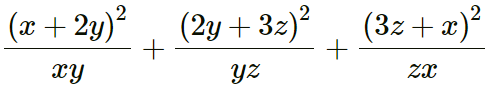Solution:

Given, x3 + 4y3 + 9z3 = 18xyz and x + 2y + 3z = 0

So,

x + 2y = – 3z, 2y + 3z = -x and 3z + x = -2y

Now,2. If a + 1/a = m and a ≠ 0; find in terms of ‘m’; the value of:

(i) a – 1/a

(ii) a2 – 1/a2

Solution:

(i) Given, a + 1/a = m

On squaring on both sides, we get

(a + 1/a)2 = m2

a2 + 1/a2 + 2 = m2

a2 + 1/a2 = m2 – 2 … (1)

Now, consider the expansion

(a – 1/a)2 = a2 + 1/a2 – 2

= m2 – 2 – 2 … [From (1)]

= m2 – 4

So,

(a – 1/a) = ±√(m2 – 4) … (2)

(ii) We know that,

a2 – 1/a2 = (a – 1/a) (a + 1/a)

= m [±√(m2 – 4)]

= ±m√(m2 – 4)

3. In the expansion of (2x2 – 8) (x – 4)2; find the value of

(i) coefficient of x3

(ii) coefficient of x2

(iii) constant term

Solution:

We have, (2x2 – 8) (x – 4)2

= (2x2 – 8) (x2 – 2 × 4 × x + 42)

= (2x2 – 8) (x2 – 8x + 16)

= 2x2(x2 – 8x + 16) – 8(x2 – 8x + 16)

= 4x4 – 16x3 + 32x2 – 8x2 + 64x – 128

= 4x4 – 16x3 + 24x2 + 64x – 128

Now,

(i) coefficient of x3 = -16

(ii) coefficient of x2 = 24

(iii) constant term = -128

4. If x > 0 and x2 + 1/9x2 = 25/36. Find: x3 + 1/27x3

Solution:

Given, x2 + 1/9x2 = 25/36 … (1)

Now, consider the expansion

(x + 1/3x)2 = x2 + (1/3x)2 + (2 × x × 1/3x)

= (x2 + 1/9x2) + 2/3

= 25/36 + 2/3 … [From (1)]

= 49/36

So,

(x + 1/3x) = ±√(49/36)

= ±7/6 … (2)

Now, consider the expansion

(x + 1/3x)3 = x3 + (1/3x)3 + 3(x + 1/3x)

(7/6)3 = x3 + (1/3x)3 + 3(7/6) …… [From (2)]

343/216 = x3 + 1/27x3 + 21/6

x3 + 1/27x3 = 343/216 – 21/6

= (343 – 252)/216

= 91/216

Thus, x3 + 1/27x3 = 91/216

5. If 2(x2 + 1) = 5x, find:

(i) x – 1/x

(ii) x3 – 1/x3

Solution:

(i) Given, 2(x2 + 1) = 5x

x2 + 1 = 5x/2

On dividing by x on both sides, we have

(x2 + 1)/x = 5/2

⇒ (x + 1/x) = 5/2 … (1)

Now, consider the expansion of (x + 1/x)2

(x + 1/x)2 = x2 + 1/x2 + 2

(5/2)2 = x2 + 1/x2 + 2 … [From (1)]

x2 + 1/x2 = 25/4 – 2

= (25 – 8)/4

= 17/4 … (2)

Now,

(x – 1/x)2 = x2 + 1/x2 – 2

= 17/4 – 2 … [From (2)]

= (17 – 8)/4

= 9/4

So,

x – 1/x = √9/4

Thus,

(i) x – 1/x = ±3/2 … (3)

Next, we know that

(x3 – 1/x3) = (x – 1/x)3 + 3(x – 1/x)

= (±3/2)3 + 3(±3/2) … [From (3)]

= ± 27/8 ± 9/2

= ± (27 + 36)/8

= ± 63/8

(ii) Thus, x3 – 1/x3 = ±63/8

6. If a2 + b2 = 34 and ab = 12; find:

(i) 3(a + b)2 + 5(a – b)2

(ii) 7(a – b)2 – 2(a + b)2

Solution:

We have, a2 + b2 = 34 and ab= 12

We know that,

(a + b)2 = (a2 + b2) + 2ab

= 34 + 2 x 12

= 34 + 24

= 58

Also, we know that

(a – b)2 = (a2 + b2) – 2ab

= 34 – 2 x 12

= 34- 24

= 10

(i) 3(a + b)+ 5(a – b)2

= 3 x 58 + 5 x 10

= 174 + 50

= 224

(ii) 7(a – b)2 – 2(a + b)2

= 7 x 10 – 2 x 58

= 70 – 116

= -46

7. If 3x – 4/x = 4 and x ≠ 0; find: 27x3 – 64/x3.

Solution:

Given, 3x – 4/x = 4

Now, let’s consider the expansion of (3x – 4/x)3

(3x – 4/x)3 = 27x3 – 64/x3 – 3 × 3x × 4/x(3x – 4/x)

(4)3 = 27x3 – 64/x3 – 36(3x – 4/x)

64 = 27x3 – 64/x3 – 36(4)

64 = 27x3 – 64/x3 – 144

27x3 – 64/x3 = 144 + 64

Hence,

27x3 – 64/x3 = 208

8. If x2 + 1/x2 = 7 and x ≠ 0; find the value of: 7x3 + 8x – 7/x3 – 8/x.

Solution:

Given, x2 + 1/x2 = 7

On subtracting 2 from both sides, we get

x2 + 1/x2 – 2 = 7 – 2

(x – 1/x)2 = 5

x – 1/x = ±√5 … (1)

Now, consider

(x – 1/x)3 = x3 – 1/x3 – 3(x – 1/x)

(±√5)3 = x3 – 1/x3 – 3(±√5)

x3 – 1/x3 = (±√5)3 + 3(±√5) … (2)

Taking,

7x3 + 8x – 7/x3 – 8/x

= 7x3 – 7/x3 + 8x – 8/x

= 7(x3 – 1/x3) + 8(x – 1/x)

= 7[(±√5)3 + 3(±√5)] + 8(±√5)

= ±35√5 ± 21√5 ± 8√5

= ±64√5

9. If x = 1/(x – 5) and x ≠ 5, find x2 – 1/x2.

Solution:

Given, x = 1/(x – 5)

By cross multiplying, we have

x (x – 5) = 1

x2 – 5x = 1

x2 – 1 = 5x

Dividing both sides by x,

(x2 – 1)/x = 5

(x – 1/x) = 5 … (1)

Now,

(x – 1/x)2 = 52

x2 + 1/x2 – 2 = 25

x2 + 1/x2 = 25 + 2

= 27 … (2)

Considering the expansion (x + 1/x)2

(x + 1/x)2 = x2 + 1/x2 + 2

(x + 1/x)2 = 27 + 2 … [From (1)]

(x + 1/x)2 = 29

x + 1/x = ±√29 … (3)

We know that,

x2 – 1/x2 = (x + 1/x) (x – 1/x)

= (±√29) (5) … [From (3)]

= ±5√29

10. If x = 1/(5 – x) and x ≠ 5; find x3 + 1/x3.

Solution:

Given, x = 1/(5 – x)

By cross multiplying, we have

x (5 – x) = 1

x2 – 5x = -1

x2 + 1 = 5x

Dividing both sides by x,

(x2 + 1)/x = 5

x + 1/x = 5 … (1)

Now,

(x + 1/x)3 = x3 + 1/x3 + 3(x + 1/x)

x3 + 1/x3 = (x + 1/x)3 – 3(x + 1/x)

= 53 – 3(5)

= 125 – 15

= 110

Thus, x3 + 1/x3 = 110

11. If 3a + 5b + 4c = 0,

Show that: 27a3 + 125b3 + 64c3 = 180abc

Solution:

Given, 3a + 5b + 4c = 0

⇒ 3a + 5b = -4c

On cubing on both sides, we have

(3a + 5b)3 = (-4c)3

(3a)3 + (5b)3 + 3 x 3a x 5b (3a + 5b) = -64c3

27a3 + 125b3 + 45ab (-4c) = -64c3

27a3 + 125b3 – 180abc = -64c3

27a3 + 125b3 + 64c3 = 180abc

– Hence Proved.

12. The sum of two numbers is 7 and the sum of their cubes is 133, find the sum of their square.

Solution:

Let’s assume a and b to be the two numbers

So, a + b = 7 and a3 + b3 = 133

We know that,

(a + b)3 = a3 + b3 + 3ab (a + b)

(7)3 = 133 + 3ab (7)

343 = 133 + 21ab

21ab = 343 – 133

= 210

⇒ ab = 21

Now,

a2 + b2 = (a + b)2 – 2ab

= 72 – 2 x 10

= 49 – 20

= 29

13. In each of the following, find the value of ‘a’:

(i) 4x2 + ax + 9 = (2x + 3)2

(ii) 4x2 + ax + 9 = (2x – 3)2

(iii) 9x2 + (7a – 5)x + 25 = (3x + 5)2

Solution:

(i) 4x2 + ax + 9 = (2x + 3)2 = 4x2 + 12x + 9

On comparing coefficients of x terms, we get

ax = 12x

So,

a = 12

(ii) 4x2 + ax + 9 = (2x – 3)2 = 4x2 + 12x + 9

On comparing coefficients of x terms, we get

ax = -12x

So,

a = -12

(iii) 9x2 + (7a – 5)x + 25 = (3x + 5)2 = 9x2 + 30x + 25

On comparing coefficients of x terms, we get

(7a – 5)x = 30x

7a – 5 = 30

7a = 35

⇒ a = 5

14. If (x2 + 1)/x = 3 1/3 and x > 1; find

(i) x – 1/x

(ii) x3 – 1/x3

Solution:

Given,

(x2 + 1)/x = 3 1/3 = 10/3

x + 1/x = 10/3

On squaring on both sides, we get

(x + 1/x)2 = (10/3)2

x2 + 1/x2 + 2 = 100/9

x2 + 1/x2 = 100/9 – 2

= (100 – 18)/9

= 82/9

Now,

(x – 1/x)2 = x2 + 1/x2 – 2

= 82/9 – 2

= (82 – 18)/9

= 64/9

x – 1/x = √(64/9)

= ±8/3

On cubing both sides, we get

(x – 1/x)3 = (8/3)3

x3 – 1/x3 – 3(x – 1/x) = 512/27

x3 – 1/x3 = 3(x – 1/x) + 512/27

= 3(8/3) + 512/27

= 24/3 + 512/27

= (216 + 512)/27

= 728/27

Therefore, x3 – 1/x3 = 728/27

15. The difference between two positive numbers is 4 and the difference between their cubes is 316.

Find:

(i) Their product

(ii) The sum of their squares

Solution:

Given, difference between two positive numbers is 4

And, the difference between their cubes is 316

Let’s assume the positive numbers to be a and b

So,

a – b = 4

a– b= 316

On cubing both sides, we have

(a – b)= 64

a– b– 3ab(a – b) = 64

Also,

Given: a– b= 316

So,

316 – 64 = 3ab(4)

252 = 12ab

So,

ab = 21

Thus, the product of numbers is 21

Now,

On squaring both sides, we get

(a – b)= 16

a+ b– 2ab = 16

a+ b= 16 + 42 = 58

Thus, sum of their squares is 58.

Exercise 4(E)

1. Simplify:

(i) (x + 6)(x + 4)(x – 2)

(ii) (x – 6)(x – 4)(x + 2)

(iii) (x – 6)(x – 4)(x – 2)

(iv) (x + 6)(x – 4)(x – 2)

Solution:

Using identity:

(x + a)(x + b)(x + c) = x3 + (a + b + c)x2 + (ab + bc + ca)x + abc

(i) We have, (x + 6)(x + 4)(x – 2)

= x3 + (6 + 4 – 2)x2 + [6 × 4 + 4 × (-2) + (-2) × 6]x + 6 × 4 × (-2)

= x3 + 8x2 + (24 – 8 – 12)x – 48

= x3 + 8x2 + 4x – 48

(ii) We have, (x – 6)(x – 4)(x + 2)

= x3 + (-6 – 4 + 2)x2 + [-6 × (-4) + (-4) × 2 + 2 × (-6)]x + (-6) × (-4) × 2

= x3 – 8x2 + (24 – 8 – 12)x + 48

= x3 – 8x2 + 4x + 48

(iii) We have, (x – 6)(x – 4)(x – 2)

= x3 + (-6 – 4 – 2)x2 + [-6 × (-4) + (-4) × (-2) + (-2) × (-6)]x + (-6) × (-4) × (-2)

= x3 – 12x2 + (24 + 8 + 12)x – 48

= x3 – 12x2 + 44x – 48

(iv) We have, (x + 6)(x – 4)(x – 2)

= x3 + (6 – 4 – 2)x2 + [6 × (-4) + (-4) × (-2) + (-2) × 6]x + 6 × (-4) × (-2)

= x3 – 0x2 + (-24 + 8 – 12)x + 48

= x3 – 28x + 48

2. Simply using following identity:

(a ± b) (a2 ∓ ab + b2) = a3 ± b3

(i) (2x + 3y) (4x2 – 6xy + 9y2)

(ii) (3x – 5/x) (9x2 + 15 + 25/x2)

(iii) (a/3 – 3b) (a2 + ab + 9b2)

Solution:

(i) We have, (2x + 3y) (4x2 – 6xy + 9y2)

= (2x + 3y) [(2x)2 – (2x)(3y) + (3y)2]

= (2x)3 + (3y)3

= 8x3 + 27y3

(ii) We have, (3x – 5/x) (9x2 + 15 + 25/x2)

= (3x – 5/x) [(3x)2 + (3x)(5/x) + (5/x)2]

= (3x)3 + (5/x)3

= 27x3 + 125/x3

(iii) We have, (a/3 – 3b) (a2/9 + ab + 9b2)

= (a/3 – 3b) [(a/3)2 + (a/3)(3b) + (3b)2]

= (a/3)3 – (3b)3

= a3/27 – 27b3

3. Using suitable identity, evaluate

(i) (104)3

(ii) (97)3

Solution:

Using identity: (a ± b)3 = a3 ± b3 ± 3ab(a ± b)

(i) (104)3 = (100 + 4)3

= (100)3 + (4)3 + 3 × 100 × 4(100 + 4)

= 1000000 + 64 + 1200 × 104

= 1000000 + 64 + 124800

= 1124864

(ii) (97)= (100 – 3)3

= (100)3 – (3)3 – 3 × 100 × 3(100 – 3)

= 1000000 – 27 – 900 × 97

= 1000000 – 27 – 87300

= 912673

4. Simply: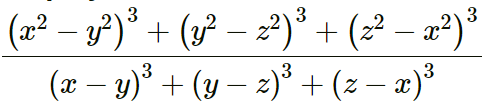Solution:

We know that,

If a + b + c = 0, then a3 + b3 + c3 = 3abc

Now, if

(x2 – y2) + (y2 – z2) + (z2 – x2) = 0

Then, we have

(x2 – y2)3 + (y2 – z2)3 + (z2 – x2)3 = 3(x2 – y2)(y2 – z2)(z2 – x2) … (1)

Similarly, if

x – y + y – z + z – x = 0

Then,

(x – y)3 + (y – z)3 + (z – x)3 = 3(x – y)(y – z)(z – x) … (2)

Now,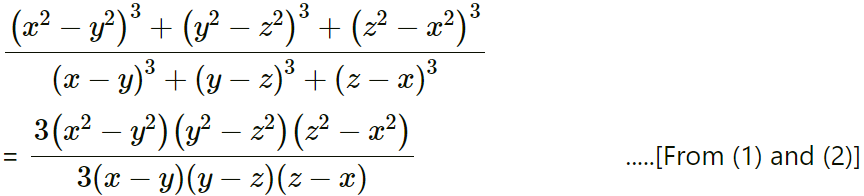= (x + y)(y + z)(z + x)

5. Evaluate:Solution:

(i) We have,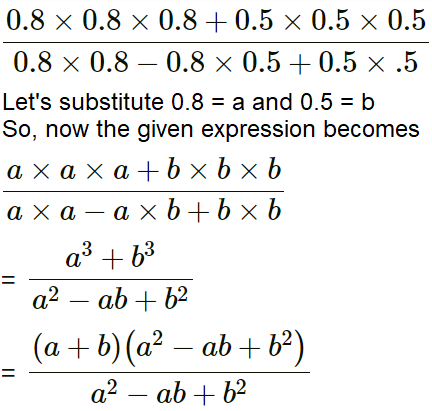= a + b

= 0.8 + 0.5

= 1.3

(ii) We have,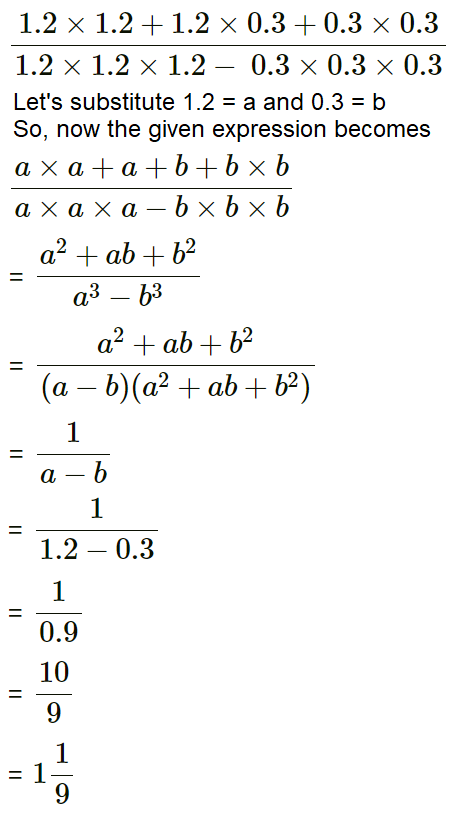6. If a – 2b + 3c = 0; state the value of a– 8b3 + 27c3.

Solution:

Given, a – 2b + 3c = 0

Then,

a– 8b3 + 27c3 = a3 + (-2b)3 + (3c)3 = 3(a)( -2b)(3c)

= -18abc

7. If x + 5y = 10; find the value of x3 + 125y3 + 150xy – 1000.

Solution:

Given, x + 5y = 10

On cubing both sides, we get

(x + 5y)3 = 103

x3 + (5y)3 + 3(x)(5y)(x + 5y) = 1000

x3 + (5y)3 + 3(x)(5y)(10) = 1000

x3 + (5y)3 + 150xy = 1000

Thus,

x3 + (5y)3 + 150xy – 1000 = 0

8. If x = 3 + 2√2, find:

(i) 1/x

(ii) x – 1/x

(iii) (x – 1/x)3

(iv) x3 – 1/x3

Solution:

We have, x = 3 + 2√2

(i) 1/x = 1/(3 + 2√2)

= (3 – 2√2)/ [(3 + 2√2) × (3 – 2√2)]

= (3 – 2√2)/ [32 – (2√2)2]

= (3 – 2√2)/ (9 – 8)

= 3 – 2√2

(ii) x – 1/x = (3 + 2√2) – (3 – 2√2) … [From (i)]

= (3 + 2√2 – 3 + 2√2)

= 4√2

(iii) (x – 1/x)3 = (4√2)3 … [From (ii)]

= (64 x 2√2)

= 128√2

(iv) (x3 – 1/x3) = (x – 1/x)3 – 3(x – 1/x)

= 128√2 – 3(4√2) … [From (iii) and (ii)]

= 128√2 – 12√2

9. If a + b = 11 and a2 + b2 = 65; find a3 + b3.

Solution:

Given, a + b = 11 and a2 + b2 = 65

Now, we know that

(a + b)2 = a2 + b2 + 2ab

(11)2 = 65 + 2ab

121 = 65 + 2ab

2ab = 121 – 65

ab = (121 – 65)/2

= 56/2

= 28

Considering the expansion (a3 + b3)

(a3 + b3) = (a + b) (a2 + b2 – ab)

= (11) (65 – 28)

= 11 × 37

= 407

Thus, a3 + b3 = 407

10. Prove that:

x2+ y+ z– xy – yz – zx is always positive.

Solution:

We have, x+ y+ z– xy – yz – zx

= 2(x+ y+ z– xy – yz – zx)

= 2x+ 2y+ 2z– 2xy – 2yz – 2zx

= x+ x2 + y+ y2 + z2 + z– 2xy – 2yz – 2zx

= (x2 + y2 – 2xy) + (z2 + x2 – 2zx) + (y2 + z2 – 2yz)

= (x – y)2 + (z – x)2 + (y – z)2

As the square of any number is positive, the given equation is always positive.

11. Find:

(i) (a + b)(a + b)

(ii) (a + b)(a + b)(a + b)

(iii) (a – b)(a – b)(a – b) by using the result of part (ii)

Solution:

(i) We have, (a + b)(a + b)

= (a + b)2

= a × a + a × b + b × a + b × b

= a2 + ab + ab + b2

= a2 + b2 + 2ab

(ii) We have, (a + b)(a + b)(a + b)

= (a × a + a × b + b × a + b × b)(a + b)

= (a2 + ab + ab + b2)(a + b)

= (a2 + b2 + 2ab)(a + b)

= a2 × a + a2 × b + b2 × a + b2 × b + 2ab × a + 2ab × b

= a3 + a2 b + ab2 + b3 + 2a2b + 2ab2

= a3 + b3 + 3a2b + 3ab2

(iii) We have, (a – b)(a – b)(a – b)

In result (ii), replacing b by -b, we get (a – b)(a – b)(a – b)

= a3 + (-b)3 + 3a2(-b) + 3a(-b)2

= a3 – b3 – 3a2b + 3ab2

## Selina Solutions for Class 9 Maths Chapter 4- Expansions

The Chapter 4, Expansions, contains 5 exercises and the Solutions given here contains the answers for all the questions present in these exercises. Let us have a look at some of the topics that are being discussed in this chapter.

4.1 Introduction

4.2 Identities

4.3 Expansions of (a+b)3

4.4 Expansions of (x+a)(x+b)

4.5 Expansions of (a+b+c)2

4.6 Using Expansions

4.7 Special Products

## Selina Solutions for Class 9 Maths Chapter 4- Expansions

In chapter 4 of Class 9, the students are taught about a process in which the contents of brackets are evaluated, the expansion. The chapter helps the students in recollecting the identities that were taught in earlier classes. An identity is an equation that is true for all values of its variables. Study the Chapter 4 of Selina textbook to understand more about Expansions. Learn the Selina Solutions for Class 9 effectively to come out with flying colours in the examinations.